8.0.0.10

### VGenerative Recursion

If you follow the design recipe of the first four parts, either you turn domain knowledge into code or you exploit the structure of the data definition to organize your code.Some functions merely compose such functions; we group those with the “structural” group. The latter functions typically decompose their arguments into their immediate structural components and then process those components. If one of these immediate components belongs to the same class of data as the input, the function is structurally recursive. While structurally designed functions make up the vast majority of code in the world, some problems cannot be solved with a structural approach to design.

To solve such complicated problems, programmers use generative recursion, a form of recursion that is strictly more powerful than structural recursion. The study of generative recursion is as old as mathematics and is often called the study of algorithms. The inputs of an algorithm represent a problem. An algorithm tends to rearrange a problem into a set of several problems, solve those, and combine their solutions into one overall solution. Often some of these newly generated problems are the same kind of problem as the given one, in which case the algorithm can be reused to solve them. In these cases, the algorithm is recursive, but its recursion uses newly generated data not immediate parts of the input data.

From the very description of generative recursion, you can tell that designing a generative recursive function is more of an ad hoc activity than designing a structurally recursive function. Still, many elements of the general design recipe apply to the design of algorithms, too, and this part of the book illustrates how and how much the design recipe helps. The key to designing algorithms is the “generation” step, which often means dividing up the problem. And figuring out a novel way of dividing a problem requires insight.In Greek, it’s “eureka!” Sometimes very little insight is required. For example, it might just require a bit of commonsense knowledge about breaking up sequences of letters. At other times, it may rely on deep mathematical theorems about numbers. In practice, programmers design simple algorithms on their own and rely on domain specialists for their complex brethren. For either kind, programmers must thoroughly understand the underlying ideas so that they can code up algorithms and have the program communicate with future readers. The best way to get acquainted with the idea is to study a wide range of examples and to develop a sense for the kinds of generative recursions that may show up in the real world.

### 25Non-standard Recursion

At this point you have designed numerous functions that employ structural recursion. When you design a function, you know you need to look at the data definition for its major input. If this input is described by a self-referential data definition, you end up with a function that refers to itself basically where the data definition refers to itself.

This chapter presents two sample programs that use recursion differently. They are illustrative of the problems that require some “eureka,” ranging from the obvious idea to the sophisticated insight.

#### 25.1Recursion without Structure

Imagine you have joined the DrRacket team. The team is working on a sharing service to support collaborations among programmers. Concretely, the next revision of DrRacket is going to enable ISL programmers to share the content of their DrRacket’s definitions area across several computers. Each time one programmer modifies the buffer, the revised DrRacket broadcasts the content of the definitions area to the instances of DrRacket that participate in the sharing session.

Sample Problem Your task is to design the function bundle, which prepares the content of the definitions area for broadcasting. DrRacket hands over the content as a list of 1Strings. The function’s task is to bundle up chunks of individual “letters” into chunks and to thus produce a list of strings—called chunksof a given length, called chunk size.

As you can see, the problem basically spells out the signature and there is no need for any problem-specific data definition:
 ; [List-of 1String] N -> [List-of String] ; bundles chunks of s into strings of length n (define (bundle s n) '())
The purpose statement reformulates a sentence fragment from the problem statement and uses the parameters from the dummy function header.

The third step calls for function examples. Here is a list of 1Strings:

(list "a" "b" "c" "d" "e" "f" "g" "h")

If we tell bundle to bundle this list into pairs—that is, n is 2then the following list is the expected result:

(list "ab" "cd" "ef" "gh")

Now if n is 3 instead, there is a left-over “letter.” Since the problem statement does not tell us which of the characters is left over, we can imagine at least two valid scenarios:
• The function produces (list "abc" "def" "g"); that is, it considers the last letter as the left-over one.

• Or, it produces (list "a" "bcd" "efg"), which packs the lead character into a string by itself.

Stop! Come up with at least one other choice.

To make things simple, we pick the first choice as the desired result and say so by writing down a corresponding test:
 (check-expect (bundle (explode "abcdefg") 3) (list "abc" "def" "g"))
Note the use of explode; it makes the test readable.

Examples and tests must also describe what happens at the boundary of data definitions. In this context, boundary clearly means bundle is given a list that is too short for the given chunk size:

(check-expect (bundle '("a" "b") 3) (list "ab"))

It also means we must consider what happens when bundle is given '(). For simplicity, we choose '() as the desired result:

(check-expect (bundle '() 3) '())

One natural alternative is to ask for '(""). Can you see others?

 ; N as compound, s considered atomic; (Processing Two Lists Simultaneously: Case 1) (define (bundle s n) (cond [(zero? n) (...)] [else (... s ... n ... (bundle s (sub1 n)))])) ; [List-of 1String] as compound, n atomic; (Processing Two Lists Simultaneously: Case 1) (define (bundle s n) (cond [(empty? s) (...)] [else (... s ... n ... (bundle (rest s) n))])) ; [List-of 1String] and N are on equal footing; (Processing Two Lists Simultaneously: Case 2) (define (bundle s n) (cond [(and (empty? s) (zero? n)) (...)] [else (... s ... n ... (bundle (rest s) (sub1 n)))])) ; consider all possibilities; (Processing Two Lists Simultaneously: Case 3) (define (bundle s n) (cond [(and (empty? s) (zero? n)) (...)] [(and (cons? s) (zero? n)) (...)] [(and (empty? s) (positive? n)) (...)] [else (... (bundle s (sub1 n)) ... ... (bundle (rest s) n) ...)]))

Figure 146: Useless templates for breaking up strings into chunks

The template step reveals that a structural approach cannot work. Figure 146 shows four possible templates. Since both arguments to bundle are complex, the first two consider one of the arguments atomic. That clearly cannot be the case because the function has to take apart each argument. The third template is based on the assumption that the two arguments are processed in lockstep, which is close—except that bundle clearly has to reset the chunk size to its original value at regular intervals. The final template says that the two arguments are processed independently, meaning there are four possibilities to proceed at each stage. This final design decouples the arguments too much because the list and the counting number must be processed together. In short, we must admit that the structural templates appear to be useless for this design problem.

 ; [List-of 1String] N -> [List-of String] ; bundles chunks of s into strings of length n ; idea take n items and drop n at a time (define (bundle s n) (cond [(empty? s) '()] [else (cons (implode (take s n)) (bundle (drop s n) n))])) ; [List-of X] N -> [List-of X] ; keeps the first n items from l if possible or everything (define (take l n) (cond [(zero? n) '()] [(empty? l) '()] [else (cons (first l) (take (rest l) (sub1 n)))])) ; [List-of X] N -> [List-of X] ; removes the first n items from l if possible or everything (define (drop l n) (cond [(zero? n) l] [(empty? l) l] [else (drop (rest l) (sub1 n))]))

Figure 147: Generative recursion

Figure 147 shows a complete definition for bundle. The definition uses the drop and take functions requested in exercise 395; these functions are also available in standard libraries. For completeness, the figure comes with their definitions: drop eliminates up to n items from the front of the list, take returns up to that many items. Using these functions, it is quite straightforward to define bundle:
1. if the given list is '(), the result is '() as decided upon;

2. otherwise bundle uses take to grab the first n 1Strings from s and implodes them into a plain String;

3. it then recurs with a list that is shortened by n items, which is accomplished with drop; and

4. finally, cons combines the string from 2 with the list of strings from 3 to create the result for the complete list.

List item 3 highlights the key difference between bundle and any function in the first four parts of this book. Because the definition of List-of conses an item onto a list to create another one, all functions in the first four parts use first and rest to deconstruct a non-empty list. In contrast, bundle uses drop, which removes not just one but n items at once.

While the definition of bundle is unusual, the underlying ideas are intuitive and not too different from the functions seen so far. Indeed, if the chunk size n is 1, bundle specializes to a structurally recursive definition. Also, drop is guaranteed to produce an integral part of the given list, not some arbitrarily rearranged version. And this idea is precisely what the next section presents.

Exercise 421. Is (bundle '("a" "b" "c") 0) a proper use of the bundle function? What does it produce? Why?

Exercise 422. Define the function list->chunks. It consumes a list l of arbitrary data and a natural number n. The function’s result is a list of list chunks of size n. Each chunk represents a sub-sequence of items in l.

Use list->chunks to define bundle via function composition.

Exercise 423. Define partition. It consumes a String s and a natural number n. The function produces a list of string chunks of size n.

For non-empty strings s and positive natural numbers n,

(equal? (partition s n) (bundle (explode s) n))

is #true. But don’t use this equality as the definition for partition; use substring instead.

Hint Have partition produce its natural result for the empty string. For the case where n is 0, see exercise 421.

Note The partition function is somewhat closer to what a cooperative DrRacket environment would need than bundle.

#### 25.2Recursion that Ignores Structure

Recall that the sort> function from Design by Composition consumes a list of numbers and rearranges it in some order, typically ascending or descending. It proceeds by inserting the first number into the appropriate position of the sorted rest of the list. Put differently, it is a structurally recursive function that reprocesses the result of the natural recursions.

Hoare’s quick-sort algorithm goes about sorting lists in a radically different manner and has become the classic example of generative recursion. The underlying generative step uses the time-honored strategy of divide-and-conquer. That is, it divides the nontrivial instances of the problem into two smaller, related problems; solves those smaller problems; and combines their solutions into a solution for the original problem. In the case of the quick-sort algorithm, the intermediate goal is to divide the list of numbers into two lists:
• one that contains all the numbers that are strictly smaller than the first

• and another one with all those items that are strictly larger.

Then the two smaller lists are sorted via the quick-sort algorithm. Once the two lists are sorted, the results are composed with the first item placed in the middle. Owing to its special role, the first item on the list is called the pivot item.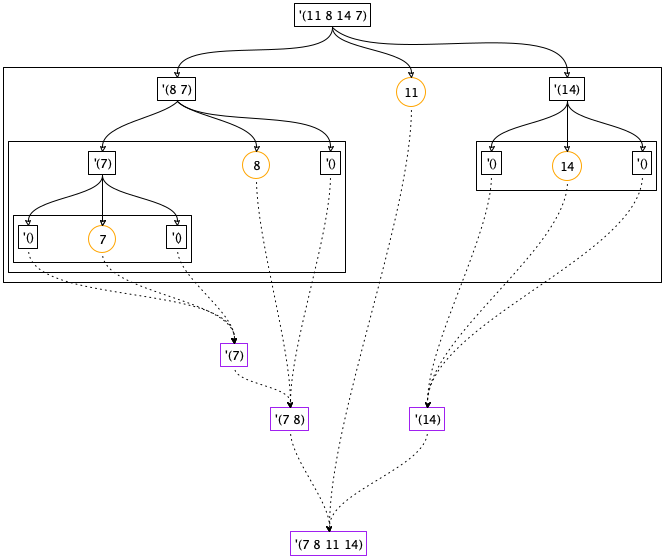Figure 148: A graphical illustration of the quick-sort algorithm

To develop an understanding of how the quick-sort algorithm works, let’s walk through an example, quick-sorting (list 11 8 14 7). Figure 148 illustrates the process in a graphical way. The figure consists of a top half, the divide phase, and the bottom half, the conquer phase.

The partition phase is represented with boxes and solid arrows. Three arrows emerge from each boxed list and go to a box with three pieces: the circled pivot element in the middle, to its left the boxed list of numbers smaller than the pivot, and to its right the boxed list of those numbers that are larger than the pivot. Each of these steps isolates at least one number as the pivot, meaning the two neighboring lists are shorter than the given list. Consequently, the overall process terminates too.

Consider the first step where the input is (list 11 8 14 7). The pivot item is 11. Partitioning the list into items larger and smaller than 11 produces (list 8 7) and (list 14). The remaining steps of the partitioning phase work in an analogous way. Partitioning ends when all numbers have been isolated as pivot elements. At this point, you can already read off the final result by reading the pivots from left to right.

The conquering phase is represented with dashed arrows and boxed lists. Three arrows enter each result box: the middle one from a pivot, the left one from the boxed result of sorting the smaller numbers, and the right one from the boxed result of sorting the larger ones. Each step adds at least one number to the result list, the pivot, meaning the lists grow toward the bottom of the diagram. The box at the bottom is a sorted variant of the given list at the top.

Take a look at the left-most, upper-most conquer step. It combines the pivot 7 with two empty lists, resulting in '(7). The next one down corresponds to the partitioning step that isolated 8 and thus yields '(7 8). Each level in the conquering phase mirrors a corresponding level from the partitioning phase. After all, the overall process is recursive.

Exercise 424. Draw a quick-sort diagram like the one in figure 148 for (list 11 9 2 18 12 14 4 1).

Now that we have a good understanding of the quick-sort idea, we can translate it into ISL+. Clearly, quick-sort< distinguishes two cases. If the input is '(), it produces '() because this list is sorted already; otherwise, it performs a generative recursion. This case split suggests the following cond expression:
 ; [List-of Number] -> [List-of Number] ; produces a sorted version of alon (define (quick-sort< alon) (cond [(empty? alon) '()] [else ...]))
The answer for the first case is given. For the second case, when quick-sort<’s input is a non-empty list, the algorithm uses the first item to partition the rest of the list into two sublists: a list with all items smaller than the pivot item and another one with those larger than the pivot item.

Since the rest of the list is of unknown size, we leave the task of partitioning the list to two auxiliary functions: smallers and largers. They process the list and filter out those items that are smaller and larger, respectively, than the pivot. Hence each auxiliary function accepts two arguments, namely, a list of numbers and a number. Designing these two functions is an exercise in structural recursion. Try on your own or read the definitions shown in figure 149.

 ; [List-of Number] -> [List-of Number] ; produces a sorted version of alon ; assume the numbers are all distinct (define (quick-sort< alon) (cond [(empty? alon) '()] [else (local ((define pivot (first alon))) (append (quick-sort< (smallers alon pivot)) (list pivot) (quick-sort< (largers alon pivot))))])) ; [List-of Number] Number -> [List-of Number] (define (largers alon n) (cond [(empty? alon) '()] [else (if (> (first alon) n) (cons (first alon) (largers (rest alon) n)) (largers (rest alon) n))])) ; [List-of Number] Number -> [List-of Number] (define (smallers alon n) (cond [(empty? alon) '()] [else (if (< (first alon) n) (cons (first alon) (smallers (rest alon) n)) (smallers (rest alon) n))]))

Figure 149: The quick-sort algorithm

Each of these lists is sorted separately, using quick-sort<, which implies the use of recursion, specifically the following two expressions:
1. (quick-sort< (smallers alon pivot)), which sorts the list of items smaller than the pivot; and

2. (quick-sort< (largers alon pivot)), which sorts the list of items larger than the pivot.

Once quick-sort< has the sorted versions of the two lists, it must combine the two lists and the pivot in the proper order: first all those items smaller than pivot, then pivot, and finally all those that are larger. Since the first and last list are already sorted, quick-sort< can simply use append:
 (append (quick-sort< (smallers alon pivot)) (list (first alon)) (quick-sort< (largers alon pivot)))
Figure 149 contains the full program; read it before proceeding.

Now that we have an actual function definition, we can evaluate the example from above by hand:
 (quick-sort< (list 11 8 14 7)) == (append (quick-sort< (list 8 7)) (list 11) (quick-sort< (list 14))) == (append (append (quick-sort< (list 7)) (list 8) (quick-sort< '())) (list 11) (quick-sort< (list 14))) == (append (append (append (quick-sort< '()) (list 7) (quick-sort< '())) (list 8) (quick-sort< '())) (list 11) (quick-sort< (list 14))) == (append (append (append '() (list 7) '()) (list 8) '()) (list 11) (quick-sort< (list 14))) == (append (append (list 7) (list 8) '()) (list 11) (quick-sort< (list 14))) ...
The calculation shows the essential steps of the sorting process, that is, the partitioning steps, the recursive sorting steps, and the concatenation of the three parts. From this calculation, it is easy to see how quick-sort< implements the process illustrated in figure 148.

Both figure 148 and the calculation also show how quick-sort< completely ignores the structure of the given list. The first recursion works on two distant numbers from the originally given list and the second one on the list’s third item. These recursions aren’t random, but they are certainly not relying on the structure of the data definition.

Contrast quick-sort<’s organization with that of the sort> function from Design by Composition. The design of the latter follows the structural design recipe, yielding a program that processes a list item by item. By splitting the list, quick-sort< can speed up the process of sorting the list, though at the cost of not using plain first and rest.

Exercise 425. Articulate purpose statements for smallers and largers in figure 149.

Exercise 426. Complete the hand-evaluation from above. A close inspection of the evaluation suggests an additional trivial case for quick-sort<. Every time quick-sort< consumes a list of one item, it returns it as is. After all, the sorted version of a list of one item is the list itself.

Modify quick-sort< to take advantage of this observation. Evaluate the example again. How many steps does the revised algorithm save?

Exercise 427. While quick-sort< quickly reduces the size of the problem in many cases, it is inappropriately slow for small problems. Hence people use quick-sort< to reduce the size of the problem and switch to a different sort function when the list is small enough.

Develop a version of quick-sort< that uses sort< (an appropriately adapted variant of sort> from Auxiliary Functions that Recur) if the length of the input is below some threshold.

Exercise 428. If the input to quick-sort< contains the same number several times, the algorithm returns a list that is strictly shorter than the input. Why? Fix the problem so that the output is as long as the input.

Exercise 429. Use filter to define smallers and largers.

Exercise 430. Develop a variant of quick-sort< that uses only one comparison function, say, <. Its partitioning step divides the given list alon into a list that contains the items of alon smaller than the pivot and another one with those that are not smaller.

Use local to package up the program as a single function. Abstract this function so that it consumes a list and a comparison function.

### 26Designing Algorithms

The overview for this part already explains that the design of generative recursion functions is more ad hoc than structural design. As the first chapter shows, two generative recursions can radically differ in how they process functions. Both bundle and quick-sort< process lists, but while the former at least respects the sequencing in the given list, the latter rearranges its given list at will. The question is whether a single design recipe can help with the creation of such widely differing functions.

The first section shows how to adapt the process dimension of the design recipe to generative recursion. The second section homes in on another new phenomenon: an algorithm may fail to produce an answer for some of its inputs. Programmers must therefore analyze their programs and supplement the design information with a comment on termination. The remaining sections contrast structural and generative recursion.

Let’s examine the six general steps of our structural design recipe in light of the examples in the preceding chapter:
• As before, we must represent the problem information as data in our chosen programming language. The choice of a data representation for a problem affects our thinking about the computational process, so some planning ahead is necessary. Alternatively, be prepared to backtrack and to explore different data representations. Regardless, we must analyze the problem information and define data collections.

• We also need a signature, a function header, and a purpose statement. Since the generative step has no connection to the structure of the data definition, the purpose statement must go beyond what the function is to compute and also explain how the function computes its result.

• It is useful to explain the “how” with function examples, the way we explained bundle and quick-sort< in the previous chapter. That is, while function examples in the structural world merely specify which output the function is to produce for which input, the purpose of examples in the world of generative recursion is to explain the underlying idea behind the computational process.

For bundle, the examples specify how the function acts in general and in certain boundary cases. For quick-sort<, the example in figure 148 illustrates how the function partitions the given list with respect to the pivot item. By adding such worked examples to the purpose statement, we—the designers—gain an improved understanding of the desired process, and we communicate this understanding to future readers of this code.

• Our discussion suggests a general template for algorithms. Roughly speaking, the design of an algorithm distinguishes two kinds of problems: those that are trivially solvable and those that are not.For this part of the book, “trivial” is a technical term. If a given problem is trivially solvable, an algorithm produces the matching solution. For example, the problems of sorting an empty list or a one-item list are trivially solvable. A list with many items is a nontrivial problem. For these nontrivial problems, algorithms commonly generate new problems of the same kind as the given one, solve those recursively, and combine the solutions into an overall solution.

Based on this sketch, all algorithms have roughly this organization:
 (define (generative-recursive-fun problem) (cond [(trivially-solvable? problem) (determine-solution problem)] [else (combine-solutions ... problem ... (generative-recursive-fun (generate-problem-1 problem)) ... (generative-recursive-fun (generate-problem-n problem)))]))
The original problem is occasionally needed to combine the solutions for the newly generated problems, which is why it is handed over to combine-solutions.

• This template is only a suggestive blueprint, not a definitive shape. Each piece of the template is to remind us to think about the following four questions:
• What is a trivially solvable problem?

• How are trivial solutions solved?

• How does the algorithm generate new problems that are more easily solvable than the original one? Is there one new problem that we generate or are there several?

• Is the solution of the given problem the same as the solution of (one of) the new problems? Or, do we need to combine the solutions to create a solution for the original problem? And, if so, do we need anything from the original problem data?

To define the algorithm as a function, we must express the answers to these four questions as functions and expressions in terms of the chosen data representation.

For this step, the table-driven attempt from Designing with Self-Referential Data Definitions might help again. Reconsider the quick-sort< example from Recursion that Ignores Structure. The central idea behind quick-sort< is to divide a given list into a list of smaller items and larger items and to sort those separately. Figure 150 spells out how some simple numeric examples work out for the nontrivial cases. From these examples it is straightforward to guess that the answer to the fourth question is to append the sorted list of smaller numbers, the pivot number, and the sorted list of larger numbers, which can easily be translated into code.

• Once the function is complete, it is time to test it. As before, the goal of testing is to discover and eliminate bugs.

 alon pivot sorted, smaller sorted, larger expected '(2 3 1 4) 2 '(1) '(3 4) '(1 2 3 4) '(2 0 1 4) 2 '(0 1) '(4) '(0 1 2 4) '(3 0 1 4) 3 '(0 1) '(4) '(0 1 3 4)

Figure 150: The table-based guessing approach for combining solutions

Exercise 431. Answer the four key questions for the bundle problem and the first three questions for the quick-sort< problem. How many instances of generate-problem are needed?

Exercise 432. Exercise 219 introduces the function food-create, which consumes a Posn and produces a randomly chosen Posn that is guaranteed to be distinct from the given one. First reformulate the two functions as a single definition, using local; then justify the design of food-create.

#### 26.2Termination

Generative recursion adds an entirely new aspect to computations: non-termination. A function such as bundle may never produce a value or signal an error for certain inputs. Exercise 421 asks what the result of (bundle '("a" "b" "c") 0) is, and here is an explanation of why it does not have a result:
 (bundle '("a" "b" "c") 0) == (cons (implode (take  '("a" "b" "c") 0)) (bundle (drop  '("a" "b" "c") 0) 0)) == (cons (implode '()) (bundle (drop  '("a" "b" "c") 0) 0)) == (cons "" (bundle (drop  '("a" "b" "c") 0) 0)) == (cons "" (bundle '("a" "b" "c") 0))
The calculation shows how evaluating (bundle '("a" "b" "c") 0) requires having a result for the very same expression. In the context of ISL+ this means the evaluation does not stop. Computer scientists say that bundle does not terminate when the second argument is 0; they also say that the function loops or that the computation is stuck in an infinite loop.

Contrast this insight with the designs presented in the first four parts. Every function designed according to the recipe either produces an answer or raises an error signal for every input. After all, the recipe dictates that each natural recursion consumes an immediate piece of the input, not the input itself. Because data is constructed in a hierarchical manner, input shrinks at every stage. Eventually the function is applied to an atomic piece of data, and the recursion stops.

This reminder also explains why generative recursive functions may diverge. According to the design recipe for generative recursion, an algorithm may generate new problems without any limitations. If the design recipe required a guarantee that the new problems were “smaller” than the given one, it would terminate.The theory of computation actually shows that we must lift these restrictions eventually. But, imposing such a restriction would needlessly complicate the design of functions such as bundle.

In this book, we therefore keep the first six steps of the design recipe mostly intact and supplement them with a seventh step: the termination argument. Figure 151 presents the first part of the design recipe for generative recursion, and figure 152 the second one. They show the design recipe in the conventional tabular form. The unmodified steps come with a dash in the activity column. Others come with comments on how the design recipe for generative recursion differs from the one for structural recursion. The last row in figure 152 is completely new.

A termination argument comes in one of two forms. The first one argues why each recursive call works on a problem that is smaller than the given one. Often this argument is straightforward; on rare occasions, you will need to work with a mathematician to prove a theorem for such arguments. The second kind illustrates with an example that the function may not terminate.You cannot define a predicate for this class; otherwise you could modify the function and ensure that it always terminates. Ideally it should also describe the class of data for which the function may loop. In rare cases, you may not be able to make either argument because computer science does not know enough yet.

 steps outcome activity problem analysis data representation and definition — header a purpose statement concerning the “how” of the function supplement the explanation of what the function computes with a one-liner on how it computes the result examples examples and tests work through the “how” with several examples template fixed template —

Figure 151: Designing algorithms (part 1)

 steps outcome activity definition full-fledged function definition formulate conditions for trivially solvable problems; formulate answers for these trivial cases; determine how to generate new problems for nontrivial problems, possibly using auxiliary functions; determine how to combine the solutions of the generated problems into a solution for the given problem tests discover mistakes — termination (1) a size argument for each recursive call or (2) examples of exceptions to termination investigate whether the problem data for each recursive data is smaller than the given data; find examples that cause the function to loop

Figure 152: Designing algorithms (part 2)

Let’s illustrate the two kinds of termination arguments with examples. For the bundle function, it suffices to warn readers about chunk size 0:
 ; [List-of 1String] N -> [List-of String] ; bundles sub-sequences of s into strings of length n ; termination (bundle s 0) loops unless s is '() (define (bundle s n) ...)
In this case, it is possible to define a predicate that precisely describes when bundle terminates. For quick-sort<, the key observation is that each recursive use of quick-sort< receives a list that is shorter than alon:
 ; [List-of Number] -> [List-of Number] ; creates a sorted variant of alon ; termination both recursive calls to quick-sort< ; receive list that miss the pivot item (define (quick-sort< alon) ...)
In one case, the list consists of the numbers that are strictly smaller than the pivot; the other one is for numbers strictly larger.

Exercise 433. Develop a checked version of bundle that is guaranteed to terminate for all inputs. It may signal an error for those cases where the original version loops.

Exercise 434. Consider the following definition of smallers, one of the two “problem generators” for quick-sort<:
 ; [List-of Number] Number -> [List-of Number] (define (smallers l n) (cond [(empty? l) '()] [else (if (<= (first l) n) (cons (first l) (smallers (rest l) n)) (smallers (rest l) n))]))
What can go wrong when this version is used with the quick-sort< definition from Recursion that Ignores Structure?

Exercise 435. When you worked on exercise 430 or exercise 428, you may have produced looping solutions. Similarly, exercise 434 actually reveals how brittle the termination argument is for quick-sort<. In all cases, the argument relies on the idea that smallers and largers produce lists that are maximally as long as the given list, and on our understanding that neither includes the given pivot in the result.

Based on this explanation, modify the definition of quick-sort< so that both functions receive lists that are shorter than the given one.

Exercise 436. Formulate a termination argument for food-create from exercise 432.

#### 26.3Structural versus Generative Recursion

The template for algorithms is so general that it includes structurally recursive functions. Consider the left side of figure 153. This template is specialized to deal with one trivial clause and one generative step. If we replace trivial? with empty? and generate with rest, we get a template for list-processing functions; see the right side of figure 153.

 (define (general P) (cond [(trivial? P) (solve P)] [else (combine-solutions P (general (generate P)))]))

 (define (special P) (cond [(empty? P) (solve P)] [else (combine-solutions P (special (rest P)))]))

Figure 153: From generative to structural recursion

Exercise 437. Define solve and combine-solutions so that
• special computes the length of its input,

• special negates each number on the given list of numbers, and

• special uppercases the given list of strings.

What do you conclude from these exercises?

Now you may wonder whether there is a real difference between structural recursive design and the one for generative recursion. Our answer is “it depends.” Of course, we could say that all functions using structural recursion are just special cases of generative recursion. This “everything is equal” attitude, however, is of no help if we wish to understand the process of designing functions. It confuses two kinds of design that require different forms of knowledge and that have different consequences. One relies on a systematic data analysis and not much more; the other requires a deep, often mathematical, insight into the problem-solving process itself. One leads programmers to naturally terminating functions; the other requires a termination argument. Conflating these two approaches is unhelpful.

#### 26.4Making Choices

When you interact with a function f that sorts lists of numbers, it is impossible for you to know whether f is sort< or quick-sort<. The two functions behave in an observably equivalent way.Observable equivalence is a central concept from the study of programming languages. This raises the question of which of the two a programming language should provide. More generally, when we can design a function using structural recursion and generative recursion, we must figure out which one to pick.

To illustrate the consequences of this choice, we discuss a classical example from mathematics: the problem of finding the greatest common divisor (gcd) of two positive natural numbers.John Stone suggested the greatest common divisor as a suitable example. All such numbers have 1 as divisor in common. On occasion—say, 2 and 3—this is also the only common divisor. Both 6 and 25 are numbers with several divisors:
• 6 is evenly divisible by 1, 2, 3, and 6;

• 25 is evenly divisible by 1, 5, and 25.

And yet, their greatest common divisor is 1. In contrast, 18 and 24 have many common divisors and their greatest common divisor is 6:
• 18 is evenly divisible by 1, 2, 3, 6, 9, and 18;

• 24 is evenly divisible by 1, 2, 3, 4, 6, 8, 12, and 24.

Completing the first three steps of the design recipe is straightforward:
 ; N[>= 1] N[>= 1] -> N ; finds the greatest common divisor of n and m (check-expect (gcd 6 25) 1) (check-expect (gcd 18 24) 6) (define (gcd n m) 42)
The signature specifies the inputs as natural numbers greater than or equal to 1.

From here we design both a structural and a generative recursive solution. Since this part of the book is about generative recursion, we merely present a structural solution in figure 154 and leave the design ideas to exercises. Just note that (= (remainder n i) (remainder m i) 0) encodes the idea that both n and m are “evenly divisible” by i.

 (define (gcd-structural n m) (local (; N -> N ; determines the gcd of n and m less than i (define (greatest-divisor-<= i) (cond [(= i 1) 1] [else (if (= (remainder n i) (remainder m i) 0) i (greatest-divisor-<= (- i 1)))]))) (greatest-divisor-<= (min n m))))

Figure 154: Finding the greatest common divisor via structural recursion

Exercise 438. In your words: how does greatest-divisor-<= work? Use the design recipe to find the right words. Why does the locally defined greatest-divisor-<= recur on (min n m)?

Although the design of gcd-structural is rather straightforward, it is also naive. It simply tests for every number between the smaller of n and m and 1 whether it divides both n and m evenly and returns the first such number. For small n and m, this works just fine. Consider the following example, however:

(gcd-structural 101135853 45014640)

The result is 177. To get there, gcd-structural checks the “evenly divisible” condition for 45014640, that is, it checks 45014640 - 177 remainders. Checking that many remainders—twice!—is a large effort, and even reasonably fast computers need time to complete this task.

Exercise 439. Copy gcd-structural into DrRacket and evaluate

(time (gcd-structural 101135853 45014640))

in the interactions area.

Since mathematicians recognized the inefficiency of this structural function a long time ago, they studied the problem of finding divisors in depth. The essential insight is that

for two natural numbers, L for large and S for small, the greatest common divisor is equal to the greatest common divisor of S and the remainder of L divided by S.

Here is how we can articulate this insight as an equation:

(gcd L S) == (gcd S (remainder L S))

Since (remainder L S) is smaller than both L and S, the right-hand side use of gcd consumes S first.

Here is how this insight applies to our small example:
• The given numbers are 18 and 24.

• According to the insight, they have the same gcd as 18 and 6.

• And these two have the same greatest common divisor as 6 and 0.

Now we seem stuck because 0 is unexpected. But, 0 can be evenly divided by every number, meaning we have found our answer: 6.

Working through the example not only validates the basic insight but also suggests how to turn the insight into an algorithm:
• when the smaller of the numbers is 0, we face a trivial case;

• the larger of the two numbers is the solution in the trivial case;

• generating a new problem requires one remainder operation; and

• the above equation tells us that the answer to the newly generated problem is also the answer to the originally given problem.

In short, the answers for the four design-recipe questions fall out.

 (define (gcd-generative n m) (local (; N[>= 1] N[>=1] -> N ; generative recursion ; (gcd L S) == (gcd S (remainder L S)) (define (clever-gcd L S) (cond [(= S 0) L] [else (clever-gcd S (remainder L S))]))) (clever-gcd (max m n) (min m n))))

Figure 155: Finding the greatest common divisor via generative recursion

Figure 155 presents the definition of the algorithm. The local definition introduces the workhorse of the function: clever-gcd. Its first cond line discovers the trivial case by comparing smaller to 0 and produces the matching solution. The generative step uses smaller as the new first argument and (remainder large small) as the new second argument to clever-gcd.

If we now use gcd-generative with our above example,

(gcd-generative 101135853 45014640)

we see that the response is nearly instantaneous. A hand-evaluation shows that clever-gcd recurs only nine times before it produces the solution:
 ... == (clever-gcd 101135853 45014640) == (clever-gcd 45014640 11106573) == (clever-gcd 11106573 588348) == (clever-gcd 588348 516309) == (clever-gcd 516309 72039) == (clever-gcd 72039 12036) == (clever-gcd 12036 11859) == (clever-gcd 11859 177) == (clever-gcd 177 0)
This also means that it checks only nine remainder conditions, clearly a much smaller effort than gcd-structural expends.

Exercise 440. Copy gcd-generative into the definitions area of DrRacket and evaluate

(time (gcd-generative 101135853 45014640))

in the interactions area.

You may now think that generative recursion design has discovered a much faster solution to the gcd problem, and you may conclude that generative recursion is always the right way to go. This judgment is too rash for three reasons. First, even a well-designed algorithm isn’t always faster than an equivalent structurally recursive function. For example, quick-sort< wins only for large lists; for small ones, the standard sort< function is faster. Worse, a badly designed algorithm can wreak havoc on the performance of a program. Second, it is typically easier to design a function using the recipe for structural recursion. Conversely, designing an algorithm requires an idea of how to generate new problems, a step that often requires some deep insight. Finally, programmers who read functions can easily understand structurally recursive functions, even without much documentation. The generative step of an algorithm, though, is based on a “eureka!” and, without a good explanation, is difficult to understand for future readers—and that includes older versions of yourself.

Experience shows that most functions in a program employ structural design; only a few exploit generative recursion. When we encounter a situation where a design could use the recipe for either structural or generative recursion, the best approach is to start with a structural version. If the result turns out to be too slow for the task at hand—and only then—it is time to explore the use of generative recursion.

Exercise 441. Evaluate

(quick-sort< (list 10 6 8 9 14 12 3 11 14 16 2))

by hand. Show only those lines that introduce a new recursive call to quick-sort<. How many recursive applications of quick-sort< are required? How many recursive applications of the append function? Suggest a general rule for a list of length n.

Evaluate

(quick-sort< (list 1 2 3 4 5 6 7 8 9 10 11 12 13 14))

by hand. How many recursive applications of quick-sort< are required? How many recursive applications of append? Does this contradict the first part of the exercise?

Exercise 442. Add sort< and quick-sort< to the definitions area. Run tests on the functions to ensure that they work on basic examples. Also develop create-tests, a function that creates large test cases randomly. Then explore how fast each works on various lists.

Does the experiment confirm the claim that the plain sort< function often wins over quick-sort< for short lists and vice versa?

Determine the cross-over point. Use it to build a clever-sort function that behaves like quick-sort< for large lists and like sort< for lists below this cross-over point. Compare with exercise 427.

Exercise 443. Given the header material for gcd-structural, a naive use of the design recipe might use the following template or some variant:
 (define (gcd-structural n m) (cond [(and (= n 1) (= m 1)) ...] [(and (> n 1) (= m 1)) ...] [(and (= n 1) (> m 1)) ...] [else (... (gcd-structural (sub1 n) (sub1 m)) ... ... (gcd-structural (sub1 n) m) ... ... (gcd-structural n (sub1 m)) ...)]))
Why is it impossible to find a divisor with this strategy?

Exercise 444. Exercise 443 means that the design for gcd-structural calls for some planning and a design-by-composition approach.

The very explanation of “greatest common denominator” suggests a two-stage approach. First design a function that can compute the listIdeally, you should use sets not lists. of divisors of a natural number. Second, design a function that picks the largest common number in the list of divisors of n and the list of divisors of m. The overall function would look like this:
 (define (gcd-structural S L) (largest-common (divisors S S) (divisors S L))) ; N[>= 1] N[>= 1] -> [List-of N] ; computes the divisors of l smaller or equal to k (define (divisors k l) '()) ; [List-of N] [List-of N] -> N ; finds the largest number common to both k and l (define (largest-common k l) 1)
Why do you think divisors consumes two numbers? Why does it consume S as the first argument in both uses?

### 27Variations on the Theme

The design of an algorithm starts with an informal description of a process of how to create a problem that is more easily solvable than the given one and whose solution contributes to the solution of the given problem. Coming up with this kind of idea requires inspiration, immersion in an application domain, and experience with many different kinds of examples.

This chapter presents several illustrative examples of algorithms. Some are directly drawn from mathematics, which is the source of many ideas; others come from computational settings. The first example is a graphical illustration of our principle: the Sierpinski triangle. The second one explains the divide-and-conquer principle with the simple mathematical example of finding the root of a function. It then shows how to turn this idea into a fast algorithm for searching sequences, a widely used application. The third section concerns “parsing” of sequences of 1Strings, also a common problem in real-world programming.

#### 27.1Fractals, a First Taste

Fractals play an important role in computational geometry. Flake writes in The Computational Beauty of Nature (The MIT Press, 1998) that “geometry can be extended to account for objects with a fractional dimension. Such objects, known as fractals, come very close to capturing the richness and variety of forms found in nature. Fractals possess structural self-similarity on multiple ... scales, meaning that a piece of a fractal will often look like the whole.”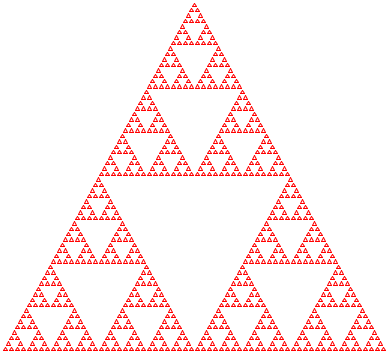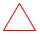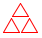Figure 156: The Sierpinski triangle

Figure 156 displays an example of a fractal shape, known as the Sierpinski triangle. The basic shape is an (equilateral) triangle, like the one in the center. When this triangle is composed sufficiently many times in a triangular fashion, we get the left-most shape.

The right-most image in figure 156 explains the generative step. When taken by itself, it says that, given a triangle, find the midpoint of each side and connect them to each other. This step yields four triangles; repeat the process for each of the outer of these three triangles unless these triangles are too small.

An alternative explanation, well suited for the shape composition functions in the 2htdp/image library,We owe this solution to Marc Smith. is based on the transition from the image in the center to the image on the right. By juxtaposing two of the center triangles and then placing one copy above these two, we also get the shape on the right:
> (s-triangle 3)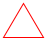> (beside (s-triangle 3) (s-triangle 3))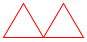> (above (s-triangle 3) (beside (s-triangle 3) (s-triangle 3)))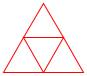This section uses the alternative description to design the Sierpinski algorithm; Accumulators as Results deals with the first description. Given that the goal is to generate the image of an equilateral triangle, we encode the problem with a (positive) number, the length of the triangle’s side. This decision yields a signature, a purpose statement, and a header:

 ; Number -> Image ; creates Sierpinski triangle of size side (define (sierpinski side) (triangle side 'outline 'red))

Now it is time to address the four questions of generative recursion:
• When the given number is so small that drawing triangles inside of it is pointless, the problem is trivial.

• In that case, it suffices to generate a triangle.

• Otherwise, the algorithm must generate a Sierpinski triangle of size side / 2 because juxtaposing two such triangles in either direction yields one of size side.

• If half-sized is the Sierpinski triangle of size side / 2, then
 (above half-sized (beside half-sized half-sized))
is a Sierpinski triangle of size side.

 (define SMALL 4) ; a size measure in terms of pixels (define small-triangle (triangle SMALL 'outline 'red)) ; Number -> Image ; generative creates Sierpinski Δ of size side by generating ; one for (/ side 2) and placing one copy above two copies (check-expect (sierpinski SMALL) small-triangle) (check-expect (sierpinski (* 2 SMALL)) (above small-triangle (beside small-triangle small-triangle))) (define (sierpinski side) (cond [(<= side SMALL) (triangle side 'outline 'red)] [else (local ((define half-sized (sierpinski (/ side 2)))) (above half-sized (beside half-sized half-sized)))]))

Figure 157: The Sierpinski algorithm

With these answers, it is straightforward to define the function. Figure 157 spells out the details. The “triviality condition” translates to (<= side SMALL) for some constant SMALL. For the trivial answer, the function returns a triangle of the given size. In the recursive case, a local expression introduces the name half-sized for the Sierpinski triangle that is half as big as the specified size. Once the recursive call has generated the small Sierpinski triangle, it composes this image via above and beside.

The figure highlights two other points. First, the purpose statement is articulated as an explanation of what the function accomplishes

; creates Sierpinski triangle of size side by ...

and how it accomplishes this goal:
 ; ... generating one of size (/ side 2) and ; placing one copy above two composed copies
Second, the examples illustrate the two possible cases: one if the given size is small enough, and one for a size that is too large still. In the latter case, the expression that computes the expected value explains exactly the meaning of the purpose statement.

Since sierpinski is based on generative recursion, defining the function and testing it is not the last step. We must also consider why the algorithm terminates for any given legal input. The input of sierpinski is a single positive number. If the number is smaller than SMALL, the algorithm terminates. Otherwise, the recursive call uses a number that is half as large as the given one. Hence, the algorithm must terminate for all positive sides, assuming SMALL is positive, too.

One view of the Sierpinski process is that it divides its problem in half until it is immediately solvable. With a little imagination, you can see that the process can be used to search for numbers with certain properties. The next section explains this idea in detail.

#### 27.2Binary Search

Applied mathematicians model the real world with nonlinear equations and then try to solve them. Specifically, they translate problems into a function f from numbers to numbers and look for some number r such that

f(r) = 0.

The value r is called the root of f.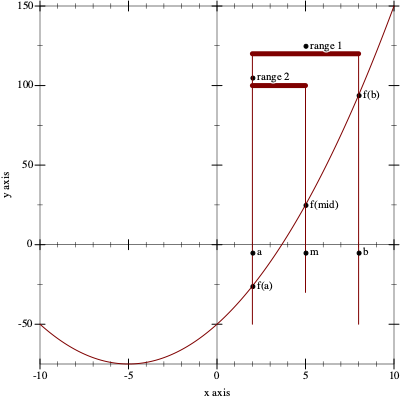Figure 158: A numeric function f with root in interval [a,b] (stage 1)

Here is a problem from the physical domain:

Sample Problem A rocket is flying at the constant speed of v miles per hour on a straight line toward some target, d0 miles away. It then accelerates at the rate of a miles per hour squared for t hours. When will it hit its target?

Physics tells us that the distance covered is the following function of time:

d(t) = (v * t + 1/2 * a * t2)

The question of when it hits the target asks us to find the time t0 such that the object reaches the desired goal:

d0 = (v * t0 + 1/2 * a * t02)

From algebra we know that this is a quadratic equation and that it is possible to solve such equations if d0, a, and v satisfy certain conditions.

Generally such problems call for more complexity than quadratic equations. In response, mathematicians have spent the last few centuries developing root-finding methods for different types of functions. In this section, we study a solution that is based on the Intermediate Value Theorem (IVT), an early result of analysis. The resulting algorithm is a primary example of generative recursion based on a mathematical theorem. Computer scientists have generalized it to the binary search algorithm.

The Intermediate Value Theorem says that a continuous function f has a root in an interval [a,b] if f(a) and f(b) are on opposite sides of the x-axis. By continuous we mean a function that doesn’t “jump,” that doesn’t have gaps, and that proceeds on a “smooth” path.

Figure 158 illustrates the Intermediate Value Theorem. The function f is a continuous function, as suggested by the uninterrupted, smooth graph. It is below the x-axis at a and above at b, and indeed, it intersects the x-axis somewhere in this interval, labeled “range 1” in the figure.

Now take a look at the midpoint between a and b:

m = (a+b) / 2

It partitions the interval [a,b] into two smaller, equally sized intervals. We can now compute the value of f at m and see whether it is below 0 or above. Here f(m) > 0, so, according to the Intermediate Value Theorem, the root is in the left interval: [a,m]. Our picture confirms this because the root is in the left half of the interval, labeled “range 2” in figure 158.

We now have a description of the key step in the root-finding process. Next, we translate this description into an ISL+ algorithm. Our first task is to state its purpose. Clearly the algorithm consumes a function and the boundaries of the interval in which we expect to find a root:
 ; [Number -> Number] Number Number -> ... (define (find-root f left right) ...)
The three parameters can’t be just any function and numbers. For find-root to work, we must assume that the following holds:
 (or (<= (f left) 0 (f right)) (<= (f right) 0 (f left)))
that is, (f left) and (f right) must be on opposite sides of the x-axis.

Next we need to fix the function’s result and formulate a purpose statement. Simply put, find-root finds an interval that contains a root. The search divides the interval until its size, (- right left), is tolerably small, say, smaller than some constant ε.DrRacket allows the use of Greek symbols such as ε. But you can also write EPSILON instead. At that point, the function could produce one of three results: the left boundary, the right one, or a representation of the interval. Any one of them completely identifies the interval, and since it is simpler to return numbers, we pick the left boundary. Here is the complete header material:
 ; [Number -> Number] Number Number -> Number ; determines R such that f has a root in [R,(+ R ε)] ; assume f is continuous ; (2) (or (<= (f left) 0 (f right)) (<= (f right) 0 (f left))) ; generative divides interval in half, the root is in ; one of the two halves, picks according to (2) (define (find-root f left right) 0)

Exercise 445. Consider the following function definition:
 ; Number -> Number (define (poly x) (* (- x 2) (- x 4)))
It defines a binomial for which we can determine its roots by hand:
 > (poly 2) 0 > (poly 4) 0
Use poly to formulate a check-satisfied test for find-root.

Also use poly to illustrate the root-finding process. Start with the interval [3,6] and tabulate the information as follows for ε = 0:
 step left f left right f right mid f mid n=1 3 -1 6.00 8.00 4.50 1.25 n=2 3 -1 4.50 1.25 ? ?

Our next task is to address the four questions of algorithm design:
1. We need a condition that describes when the problem is solved and a matching answer. Given our discussion so far, this is straightforward:

(<= (- right left) ε)

2. The matching result in the trivial case is left.

3. For the generative case, we need an expression that generates new problems for find-root. According to our informal description, this step requires determining the midpoint and its function value:
 (local ((define mid (/ (+ left right) 2)) (define [email protected] (f mid))) ...)
The midpoint is then used to pick the next interval. Following IVT, the interval [left,mid] is the next candidate if

(or (<= (f left) 0 [email protected]) (<= [email protected] 0 (f left)))

while [mid,right] is used for the recursive call if

(or (<= [email protected] 0 (f right)) (<= (f right) 0 [email protected]))

Translated into code, the body of local must be a conditional:
 (cond [(or (<= (f left) 0 [email protected]) (<= [email protected] 0 (f left))) (... (find-root f left mid) ...)] [(or (<= [email protected] 0 (f right)) (<= (f right) 0 [email protected])) (... (find-root f mid right) ...)])
In both clauses, we use find-root to continue the search.

4. The answer to the final question is obvious. Since the recursive call to find-root finds the root of f, there is nothing else to do.

The completed function is displayed in figure 159; the following exercises elaborate on its design.

 ; [Number -> Number] Number Number -> Number ; determines R such that f has a root in [R,(+ R ε)] ; assume f is continuous ; assume (or (<= (f left) 0 (f right)) (<= (f right) 0 (f left))) ; generative divides interval in half, the root is in one of the two ; halves, picks according to assumption (define (find-root f left right) (cond [(<= (- right left) ε) left] [else (local ((define mid (/ (+ left right) 2)) (define [email protected] (f mid))) (cond [(or (<= (f left) 0 [email protected]) (<= [email protected] 0 (f left))) (find-root f left mid)] [(or (<= [email protected] 0 (f right)) (<= (f right) 0 [email protected])) (find-root f mid right)]))]))

Figure 159: The find-root algorithm

Exercise 446. Add the test from exercise 445 to the program in figure 159. Experiment with different values for ε.

Exercise 447. The poly function has two roots. Use find-root with poly and an interval that contains both roots.

Exercise 448. The find-root algorithm terminates for all (continuous) f, left, and right for which the assumption holds. Why? Formulate a termination argument.

Hint Suppose the arguments of find-root describe an interval of size S1. How large is the distance between left and right for the first and second recursive call to find-root? After how many steps is (- right left) smaller than or equal to ε?

Exercise 449. As presented in figure 159, find-root computes the value of f for each boundary value twice to generate the next interval. Use local to avoid this recomputation.

In addition, find-root recomputes the value of a boundary across recursive calls. For example, (find-root f left right) computes (f left) and, if [left,mid] is chosen as the next interval, find-root computes (f left) again. Introduce a helper function that is like find-root but consumes not only left and right but also (f left) and (f right) at each recursive stage.

How many recomputations of (f left) does this design maximally avoid? Note The two additional arguments to this helper function change at each recursive stage, but the change is related to the change in the numeric arguments. These arguments are so-called accumulators, which are the topic of Accumulators.

Exercise 450. A function f is monotonically increasing if (<= (f a) (f b)) holds whenever (< a b) holds. Simplify find-root assuming the given function is not only continuous but also monotonically increasing.

Exercise 451. A table is a structure of two fields: the natural number length and a function array,Many programming languages, including Racket, support arrays and vectors, which are similar to tables. which consumes natural numbers and, for those between 0 and length (exclusive), produces answers:
 (define-struct table [length array]) ; A Table is a structure: ;   (make-table N [N -> Number])
Since this data structure is somewhat unusual, it is critical to illustrate it with examples:
 (define table1 (make-table 3 (lambda (i) i))) ; N -> Number (define (a2 i) (if (= i 0) pi (error "table2 is not defined for i =!= 0"))) (define table2 (make-table 1 a2))
Here table1’s array function is defined for more inputs than its length field allows; table2 is defined for just one input, namely 0. Finally, we also define a useful function for looking up values in tables:
 ; Table N -> Number ; looks up the ith value in array of t (define (table-ref t i) ((table-array t) i))

The root of a table t is a number in (table-array t) that is close to 0. A root index is a natural number i such that (table-ref t i) is a root of table t. A table t is monotonically increasing if (table-ref t 0) is less than (table-ref t 1), (table-ref t 1) is less than (table-ref t 2), and so on.

Design find-linear. The function consumes a monotonically increasing table and finds the smallest index for a root of the table. Use the structural recipe for N, proceeding from 0 through 1, 2, and so on to the array-length of the given table. This kind of root-finding process is often called a linear search.

Design find-binary, which also finds the smallest index for the root of a monotonically increasing table but uses generative recursion to do so. Like ordinary binary search, the algorithm narrows an interval down to the smallest possible size and then chooses the index. Don’t forget to formulate a termination argument.

Hint The key problem is that a table index is a natural number, not a plain number. Hence the interval boundary arguments for find must be natural numbers. Consider how this observation changes (1) the nature of trivially solvable problem instances, (2) the midpoint computation, (3) and the decision as to which interval to generate next. To make this concrete, imagine a table with 1024 slots and the root at 1023. How many calls to find are needed in find-linear and find-binary, respectively?

#### 27.3A Glimpse at Parsing

As mentioned in Iterative Refinement, computers come with files, which provide a form of permanent memory. From our perspective a file is just a list of 1Strings,The exact convention differs from one operating system to another, but for our purposes this is irrelevant. though interrupted by a special string:
 ; A File is one of: ; – '() ; – (cons "\n" File) ; – (cons 1String File) ; interpretation represents the content of a file ; "\n" is the newline character
The idea is that Files are broken into lines, where "\n" represents the so-called newline character, which indicates the end of a line. Let’s also introduce lines before we move on:

; A Line is a [List-of 1String].

Many functions need to process files as list of lines. The read-lines from the 2htdp/batch-io library is one of them. Concretely, the function turns the file
 (list "h" "o" "w" " " "a" "r" "e" " " "y" "o" "u" "\n" "d" "o" "i" "n" "g" "?" "\n" "a" "n" "y" " " "p" "r" "o" "g" "r" "e" "s" "s" "?")
into a list of three lines:
 (list (list "h" "o" "w" " " "a" "r" "e" " " "y" "o" "u") (list "d" "o" "i" "n" "g" "?") (list "a" "n" "y" " " "p" "r" "o" "g" "r" "e" "s" "s" "?"))
Similarly, the file

(list "a" "b" "c" "\n" "d" "e" "\n" "f" "g" "h" "\n")

also corresponds to a list of three lines:
 (list (list "a" "b" "c") (list "d" "e") (list "f" "g" "h"))
Stop! What are the list-of-lines representations for these three cases: '(), (list "\n"), and (list "\n" "\n")? Why are these examples important test cases?

The problem of turning a sequence of 1Strings into a list of lines is called the parsing problem. Many programming languages provide functions that retrieve lines, words, numbers, and other kinds of so-called tokens from files. But even if they do, it is common that programs need to parse these tokens even further. This section provides a glimpse at a parsing technique. Parsing is so complex and so central to the creation of full-fledged software applications, however, that most undergraduate curricula come with at least one course on parsing. So do not think you can tackle real parsing problems properly even after mastering this section.

We start by stating the obvious—a signature, a purpose statement, one of the above examples, and a header—for a function that turns a File into a list of Lines:
 ; File -> [List-of Line] ; converts a file into a list of lines (check-expect (file->list-of-lines (list "a" "b" "c" "\n" "d" "e" "\n" "f" "g" "h" "\n")) (list (list "a" "b" "c") (list "d" "e") (list "f" "g" "h"))) (define (file->list-of-lines afile) '())

It is also easy to describe the parsing process, given our experience with Recursion without Structure:
1. The problem is trivially solvable if the file is '().

2. In that case, the file doesn’t contain a line.

3. Otherwise, the file contains at least one "\n" or some other 1String. These items—up to and including the first "\n", if any—must be separated from the rest of the File. The remainder is a new problem of the same kind that file->list-of-lines can solve.

4. It then suffices to cons the initial segment as a single line to the list of Lines that result from the rest of the File.

The four questions suggest a straightforward instantiation of the template for generative recursive functions. Because the separation of the initial segment from the rest of the file requires a scan of an arbitrarily long list of 1Strings, we put two auxiliary functions on our wish list: first-line, which collects all 1Strings up to, but excluding, the first occurrence of "\n" or the end of the list; and remove-first-line, which removes the very same items that first-line collects.

 ; File -> [List-of Line] ; converts a file into a list of lines (define (file->list-of-lines afile) (cond [(empty? afile) '()] [else (cons (first-line afile) (file->list-of-lines (remove-first-line afile)))])) ; File -> Line ; retrieves the prefix of afile up to the first occurrence of NEWLINE (define (first-line afile) (cond [(empty? afile) '()] [(string=? (first afile) NEWLINE) '()] [else (cons (first afile) (first-line (rest afile)))])) ; File -> File ; drops the suffix of afile behind the first occurrence of NEWLINE (define (remove-first-line afile) (cond [(empty? afile) '()] [(string=? (first afile) NEWLINE) (rest afile)] [else (remove-first-line (rest afile))])) (define NEWLINE "\n") ; the 1String

Figure 160: Translating a file into a list of lines

From here, it is easy to create the rest of the program. In file->list-of-lines, the answer in the first clause must be '() because an empty file does not contain any lines. The answer in the second clause must cons the value of (first-line afile) onto the value (file->list-of-lines (remove-first-line afile)), because the first expression computes the first line and the second one computes the rest of the lines. Finally, the auxiliary functions traverse their inputs in a structurally recursive manner; their development is a straightforward exercise. Figure 160 presents the complete program code.

Here is how file->list-of-lines processes the second test:
 (file->list-of-lines (list "a" "b" "c" "\n" "d" "e" "\n" "f" "g" "h" "\n")) == (cons (list "a" "b" "c") (file->list-of-lines (list "d" "e" "\n" "f" "g" "h" "\n"))) == (cons (list "a" "b" "c") (cons (list "d" "e") (file->list-of-lines (list "f" "g" "h" "\n")))) == (cons (list "a" "b" "c") (cons (list "d" "e") (cons (list "f" "g" "h") (file->list-of-lines '())))) == (cons (list "a" "b" "c") (cons (list "d" "e") (cons (list "f" "g" "h") '())))
This evaluation is another reminder that the argument of the recursive application of file->list-of-lines is almost never the rest of the given file. It also shows why this generative recursion is guaranteed to terminate for every given File. Every recursive application consumes a list that is shorter than the given one, meaning the recursive process stops when the process reaches '().

Exercise 452. Both first-line and remove-first-line are missing purpose statements. Articulate proper statements.

Exercise 453. Design the function tokenize. It turns a Line into a list of tokens. Here a token is either a 1String or a String that consists of lower-case letters and nothing else. That is, all white-space 1Strings are dropped; all other non-letters remain as is; and all consecutive letters are bundled into “words.” Hint Read up on the string-whitespace? function.

Exercise 454. Design create-matrix. The function consumes a number n and a list of n2 numbers. It produces anmatrix, for example:
 (check-expect (create-matrix 2 (list 1 2 3 4)) (list (list 1 2) (list 3 4)))
Make up a second example.

### 28Mathematical Examples

Many solutions to mathematical problems employ generative recursion. A future programmer must get to know such solutions for two reasons. On the one hand, a fair number of programming tasks are essentially about turning these kinds of mathematical ideas into programs. On the other hand, practicing with such mathematical problems often proves inspirational for the design of algorithms. This chapter deals with three such problems.

#### 28.1Newton’s Method

Binary Search introduces one method for finding the root of a mathematical function. As the exercises in the same section sketch, the method naturally generalizes to computational problems, such as finding certain values in tables, vectors, and arrays. In mathematical applications, programmers tend to employ methods that originate from analytical mathematics. A prominent one is due to Newton. Like binary search, the so-called Newton method repeatedly improves an approximation to the root until it is “close enough.” Starting from a guess, say, r1, the essence of the process is to construct the tangent of f at r1 and to determine its root. While the tangent approximates the function, it is also straightforward to determine its root. By repeating this process sufficiently often,Newton proved this fact. an algorithm can find a root r for which (f r) is close enough to 0.

Clearly, this process relies on two pieces of domain knowledge about tangents: their slopes and roots. Informally, a tangent of f at some point r1 is the line that goes through the point (r1, f(r1)) and has the same slope as f. One mathematical way to obtain the tangent’s slope is to pick two close points on the x-axis that are equidistant from r1 and to use the slope of the line determined by f at those two points. The convention is to choose a small number ε and to work with r1 + ε and r1 - ε. That is, the points are (r1 - ε, f(r1 - ε)) and (r1 + ε, f(r1 + ε)), which determine a line and a slope: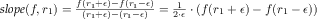Exercise 455. Translate this mathematical formula into the ISL+ function slope, which maps function f and a number r1 to the slope of f at r1. Assume that ε is a global constant. For your examples, use functions whose exact slope you can figure out, say, horizontal lines, linear functions, and perhaps polynomials if you know some calculus.

The second piece of domain knowledge concerns the root of a tangent, which is just a line or a linear function. The tangent goes through (r1, f(r1)) and has the above slope. Mathematically, it is defined as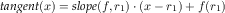Finding the root of tangent means finding a value root-of-tangent so that tangent(root-of-tangent) equals 0: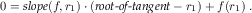We can solve this equation in a straightforward manner: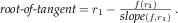Exercise 456. Design root-of-tangent, a function that maps f and r1 to the root of the tangent through (r1,(f r1)).

Now we can use the design recipe to translate the description of Newton’s process into an ISL+ program. The function—let’s call it newton in honor of its inventor—consumes a function f and a number r1:
 ; [Number -> Number] Number -> Number ; finds a number r such that (f r) is small ; generative repeatedly generates improved guesses (define (newton f r1) 1.0)

For the template of newton, we turn to the central four questions of the design recipe for generative recursion:
1. If (f r1) is close enough to 0, the problem is solved. Close to 0 could mean (f r1) is a small positive number or a small negative number. Hence we check its absolute value:

(<= (abs (f r1)) ε)

2. The solution is r1.

3. The generative step of the algorithm consists of finding the root of the tangent of f at r1, which generates the next guess. By applying newton to f and this new guess, we resume the process.

4. The answer of the recursion is also the answer of the original problem.

 ; [Number -> Number] Number -> Number ; finds a number r such that (<= (abs (f r)) ε) (check-within (newton poly 1) 2 ε) (check-within (newton poly 3.5) 4 ε) (define (newton f r1) (cond [(<= (abs (f r1)) ε) r1] [else (newton f (root-of-tangent f r1))])) ; see exercise 455 (define (slope f r) ...) ; see exercise 456 (define (root-of-tangent f r) ...)

Figure 161: The Newton process

Figure 161 displays newton. It includes two tests that are derived from the tests in Binary Search for find-root. After all, both functions search for the root of a function, and poly has two known roots.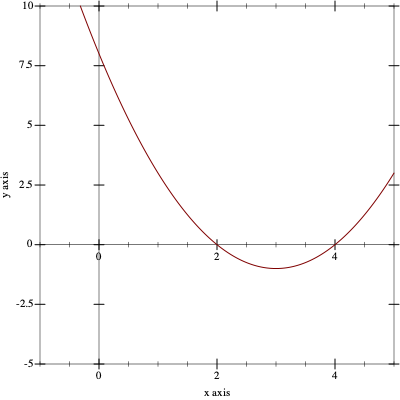Figure 162: The graph of poly on the interval [-1,5]

We are not finished with the design of newton. The new, seventh step of the design recipe calls for an investigation into the termination behavior of the function. For newton, the problem shows up with poly:
 ; Number -> Number (define (poly x) (* (- x 2) (- x 4)))
As mentioned, its roots are 2 and 4. The graph of poly in figure 162 confirms these roots and also shows that between the two roots the function flattens out. For a mathematically inclined person, this shape raises the question of what newton computes for an initial guess of 3:
 > (poly 3) -1 > (newton poly 3) /:division by zero
The explanation is that slope produces a “bad” value and the root-of-tangent function turns it into an error:
 > (slope poly 3) 0 > (root-of-tangent poly 3) /:division by zero

In addition to this run-time error, newton exhibits two other problems with respect to termination. Fortunately, we can demonstrate both with poly. The first one concerns the nature of numbers, which we briefly touched on in The Arithmetic of Numbers. It is safe to ignore the distinction between exact and inexact numbers for many beginner exercises in programming, but when it comes to translating mathematics into programs, you need to proceed with extreme caution. Consider the following:
 > (newton poly 2.9999)
An ISL+ program treats 2.9999 as an exact number, and the computations in newton process it as such, though because the numbers aren’t integers, the computation uses exact rational fractions. Since the arithmetic for fractions can get much slower than the arithmetic for inexact numbers, the above function call takes a significant amount of time in DrRacket. Depending on your computer, it may take between a few seconds and a minute or more. If you happen to choose other numbers that trigger this form of computation, it may seem as if the call to newton does not terminate at all.

The second problem concerns non-termination. Here is the example:
 > (newton poly #i3.0)
It uses the inexact number #i3.0 as the initial guess, which unlike 3 causes a different kind of problem. Specifically, the slope function now produces an inexact 0 for poly while root-of-tangent jumps to infinity:
 > (slope poly #i3.0) #i0.0 > (root-of-tangent poly #i3.0) #i+inf.0
As a result, the evaluation immediately falls into an infinite loop.The calculation in newton turns #i+inf.0 into +nan.0, a piece of data that says “not a number.” Most arithmetic operations propagate this value, which explains the behavior of newton.

In short, newton exhibits the full range of problems when it comes to complex termination behavior. For some inputs, the function produces a correct result. For some others, it signals errors. And for yet others, it goes into infinite loop or appears to go into one. The header for newtonor some other piece of writing—must warn others who wish to use the function and future readers of these complexities, and good math libraries in common programming languages do so.

Exercise 457. Design the function double-amount,This exercise was suggested by Adrian German. which computes how many months it takes to double a given amount of money when a savings account pays interest at a fixed rate on a monthly basis.

Domain Knowledge With a minor algebraic manipulation, you can show that the given amount is irrelevant. Only the interest rate matters. Also domain experts know that doubling occurs after roughly 72/r month as long as the interest rate r is “small.”

#### 28.2Numeric Integration

Many physics problems boil down to determining the area under a curve:

Sample Problem A car drives at a constant speed of v meters per second. How far does it travel in 5, 10, 15 seconds?

A rocket lifts off at the constant rate of acceleration of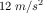. What height does it reach after 5, 10, 15 seconds?

Physics tells us that a vehicle travels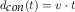meters if it moves at a constant speed v for t seconds. For vehicles that accelerate, the distance traveled depends on the square of the time t passed: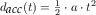In general, the law tells us that the distance corresponds to the area under the graph of speed v(t) over time t.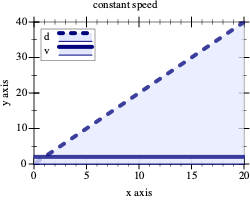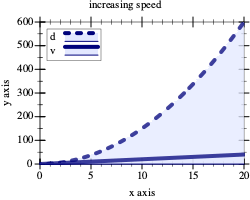Figure 163: Distance traveled with constant vs accelerating speed

Figure 163 illustrates the idea in a graphical manner. On the left, we see an overlay of two graphs: the solid flat line is the speed of the vehicle and the rising dashed line is the distance traveled. A quick check shows that the latter is indeed the area determined by the former and the x-axis at every point in time. Similarly, the graphs on the right show the relationship between a rocket moving at constantly increasing speed and the height it reaches. Determining this area under the graph of a function for some specific interval is called (function) integration.

While mathematicians know formulas for the two sample problems that give precise answers, the general problem calls for computational solutions. The problem is that curves often come with complex shapes, more like those in figure 164, which suggests that someone needs to know the area between the x-axis, the vertical lines labeled a and b, and the graph of f. Applied mathematicians determine such areas in an approximate manner, summing the areas of many small geometric shapes. It is therefore natural to develop algorithms that deal with these calculations.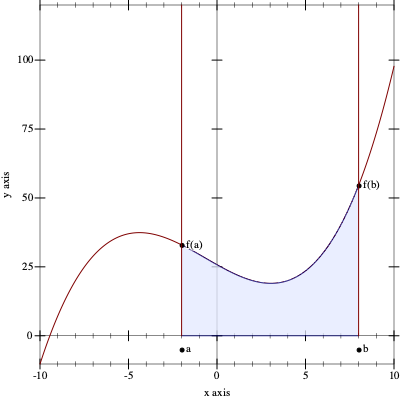Figure 164: Integrating a function f between a and b

An integration algorithm consumes three inputs: the function f and two borders, a and b. The fourth part, the x-axis, is implied. This suggests the following signature:
In order to understand the idea behind integration, it is best to study simple examples such as a constant function or a linear one. Thus, consider

(define (constant x) 20)

Passing constant to integrate, together with 12 and 22, describes a rectangle of width 10 and height 20. The area of this rectangle is 200, meaning we get this test:

(check-expect (integrate constant 12 22) 200)

Similarly, let’s use linear to create a second test:

(define (linear x) (* 2 x))

If we use linear, 0, and 10 with integrate, the area is a triangle with a base width of 10 and a height of 20. Here is the example as a test:

(check-expect (integrate linear 0 10) 100)

After all, a triangle’s area is half of the product of its base width and height.

For a third example, we exploit some domain-specific knowledge. As mentioned, mathematicians know how to determine the area under some functions in a precise manner. For example, the area under the function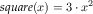on the interval [a,b] can be calculated with the following formula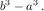Here is how to turn this idea into a concrete test:
 (define (square x) (* 3 (sqr x))) (check-expect (integrate square 0 10) (- (expt 10 3) (expt 0 3)))

 (define ε 0.1) ; [Number -> Number] Number Number -> Number ; computes the area under the graph of f between a and b ; assume (< a b) holds (check-within (integrate (lambda (x) 20) 12 22) 200 ε) (check-within (integrate (lambda (x) (* 2 x)) 0 10) 100 ε) (check-within (integrate (lambda (x) (* 3 (sqr x))) 0 10) 1000 ε) (define (integrate f a b) #i0.0)

Figure 165: A generic integration function

Figure 165 collects the result of the first three steps of the design recipe. The figure adds a purpose statement and an obvious assumption concerning the two interval boundaries. Instead of check-expect it uses check-within, which anticipates the numerical inaccuracies that come with computational approximations in such calculations. Analogously, the header of integrate specifies #i0.0 as the return result, signaling that the function is expected to return an inexact number.

The following two exercises show how to turn domain knowledge into integration functions. Both functions compute rather crude approximations. While the design of the first uses only mathematical formulas, the second also exploits a bit of structural design ideas. Solving these exercises creates the necessary appreciation for the core of this section, which presents a generative-recursive integration algorithm.

Exercise 458. Kepler suggested a simple integration method. To compute an estimateThe method is known as Kepler’s rule. of the area under f between a and b, proceed as follows:
1. divide the interval into half at mid = (a + b) / 2;

2. compute the areas of these two trapezoids:
• [(a,0),(a,f(a)),(mid,0),(mid,f(mid))]

• [(mid,0),(mid,f(mid)),(b,0),(b,f(b))];

3. then add the two areas.

Domain Knowledge Let’s take a look at these trapezoids. Here are the two possible shapes, with minimal annotations to reduce clutter: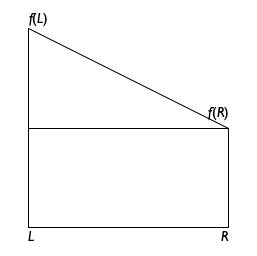The left shape assumes f(L) > f(R) while the right one shows the case where f(L) < f (R). Despite the asymmetry, it is still possible to calculate the area of these trapezoids with a single formula: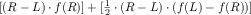Stop! Convince yourself that this formula adds the area of the triangle to the area of the lower rectangle for the left trapezoid, while it subtracts the triangle from the area of the large rectangle for the right one.

Also show that the above formula is equal to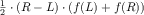This is a mathematical validation of the asymmetry of the formula.

Design the function integrate-kepler. That is, turn the mathematical knowledge into an ISL+ function. Adapt the test cases from figure 165 to this use. Which of the three tests fails and by how much?

Exercise 459. Another simple integration method divides the area into many small rectangles. Each rectangle has a fixed width and is as tall as the function graph in the middle of the rectangle. Adding up the areas of the rectangles produces an estimate of the area under the function’s graph.

Let’s use

R = 10

to stand for the number of rectangles to be considered. Hence the width of each rectangle isThe height of one of these rectangles is the value of f at its midpoint. The first midpoint is clearly at a plus half of the width of the rectangle,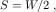which means its area is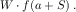To compute the area of the second rectangle, we must add the width of one rectangle to the first midpoint: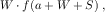For the third one, we get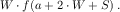In general, we can use the following formula for the ith rectangle: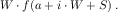The first rectangle has index 0, the last one R - 1.

Using these rectangles, we can now determine the area under the graph: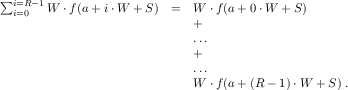Turn the description of the process into an ISL+ function. Adapt the test cases from figure 165 to this case.

The more rectangles the algorithm uses, the closer its estimate is to the actual area. Make R a top-level constant and increase it by factors of 10 until the algorithm’s accuracy eliminates problems with an ε value of 0.1.

Decrease ε to 0.01 and increase R enough to eliminate any failing test cases again. Compare the result to exercise 458.

The Kepler method of exercise 458 immediately suggests a divide-and-conquer strategy like binary search introduced in Binary Search. Roughly speaking, the algorithm would split the interval into two pieces, recursively compute the area of each piece, and add the two results.

Exercise 460. Develop the algorithm integrate-dc, which integrates a function f between the boundaries a and b using a divide-and-conquer strategy. Use Kepler’s method when the interval is sufficiently small.

The divide-and-conquer approach of exercise 460 is wasteful. Consider a function whose graph is level in one part and rapidly changes in another; see figure 166 for a concrete example. For the level part on the graph, it is pointless to keep splitting the interval. It is just as easy to compute the trapezoid for the complete interval as for the two halves. For the “wavy” part, however, the algorithm must continue dividing the interval until the irregularities of the graph are reasonably small.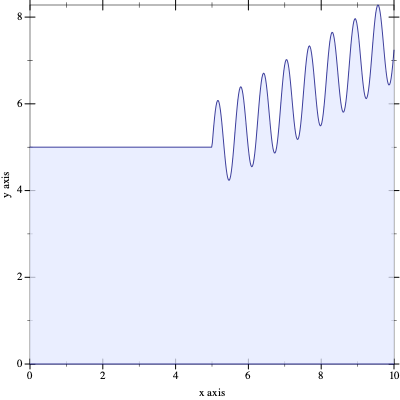Figure 166: A candidate for adaptive integration

To discover when f is level, we can change the algorithm as follows. Instead of just testing how large the interval is, the new algorithm computes the area of three trapezoids: the given one and the two halves. If the difference between the two is less than the area of a small rectangle of height ε and width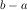,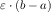it is safe to assume that the overall area is a good approximation. In other words, the algorithm determines whether f changes so much that it affects the error margin. If so, it continues with the divide-and-conquer approach; otherwise it stops and uses the Kepler approximation.

Exercise 461. Design integrate-adaptive. That is, turn the recursive process description into an ISL+ algorithm. Make sure to adapt the test cases from figure 165 to this use.

Do not discuss the termination of integrate-adaptive.

Does integrate-adaptive always compute a better answer than either integrate-kepler or integrate-rectangles? Which aspect is integrate-adaptive guaranteed to improve?

Terminology The algorithm is called adaptive integration because it automatically allocates time to those parts of the graph that need it and spends little time on the others. Specifically, for those parts of f that are level, it performs just a few calculations; for the other parts, it inspects small intervals to decrease the error margin. Computer science knows many adaptive algorithms, and integrate-adaptive is just one of them.

#### 28.3Project: Gaussian Elimination

Mathematicians not only search for solutions of equations in one variable; they also study whole systems of linear equations:

Sample Problem In a bartering world, the values of coal (x), oil (y), and gas (z) are determined by these exchange equations: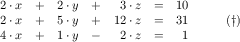A solution to such a system of equations consists of a collection of numbers, one per variable, such that if we replace the variable with its corresponding number, the two sides of each equation evaluate to the same number. In our running example, the solution is

x = 1, y = 1, and z = 2.

We can easily check this claim: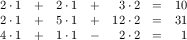The three equations reduce to

10 = 10, 31 = 31, and 1 =1.

 ; An SOE is a non-empty Matrix. ; constraint for (list r1 ... rn), (length ri) is (+ n 1) ; interpretation represents a system of linear equations ; An Equation is a [List-of Number]. ; constraint an Equation contains at least two numbers. ; interpretation if (list a1 ... an b) is an Equation, ; a1, ..., an are the left-hand-side variable coefficients ; and b is the right-hand side ; A Solution is a [List-of Number] (define M ; an SOE (list (list 2 2  3 10) ; an Equation (list 2 5 12 31) (list 4 1 -2  1))) (define S '(1 1 2)) ; a Solution

Figure 167: A data representation for systems of equations

Figure 167 introduces a data representation for our problem domain. It includes an example of a system of equations and its solution. This representation captures the essence of a system of equations, namely, the numeric coefficients of the variables on the left-hand side and the right-hand-side values. The names of the variables don’t play any role because they are like parameters of functions; meaning, as long as they are consistently renamed the equations have the same solutions.

For the rest of this section, it is convenient to use these functions:
 ; Equation -> [List-of Number] ; extracts the left-hand side from a row in a matrix (check-expect (lhs (first M)) '(2 2 3)) (define (lhs e) (reverse (rest (reverse e)))) ; Equation -> Number ; extracts the right-hand side from a row in a matrix (check-expect (rhs (first M)) 10) (define (rhs e) (first (reverse e)))

Exercise 462. Design the function check-solution. It consumes an SOE and a Solution. Its result is #true if plugging in the numbers from the Solution for the variables in the Equations of the SOE produces equal left-hand-side values and right-hand-side values; otherwise the function produces #false. Use check-solution to formulate tests with check-satisfied.

Hint Design the function plug-in first. It consumes the left-hand side of an Equation and a Solution and calculates out the value of the left-hand side when the numbers from the solution are plugged in for the variables.

Gaussian elimination is a standard method for finding solutions to systems of linear equations. It consists of two steps. The first step is to transform the system of equations into a system of different shape but with the same solution. The second step is to find solutions to one equation at a time. Here we focus on the first step because it is another interesting instance of generative recursion.

The first step of the Gaussian elimination algorithm is called “triangulation” because the result is a system of equations in the shape of a triangle. In contrast, the original system is a rectangle. To understand this terminology, take a look at this list, which represents the original system:
 (list (list 2 2  3 10) (list 2 5 12 31) (list 4 1 -2 1))
Triangulation transforms this matrix into the following:
 (list (list 2 2  3 10) (list   3  9 21) (list      1  2))
As promised, the shape of this system of equations is (roughly) a triangle.

Exercise 463. Check that the following system of equationshas the same solution as the one labeled with (). Do so by hand and with check-solution from exercise 462.

The key idea of triangulation is to subtract the first Equation from the remaining ones. To subtract one Equation from another means to subtract the corresponding coefficients in the two Equations. With our running example, subtracting the first equation from the second yields the following matrix:
 (list (list 2 2  3 10) (list 0 3  9 21) (list 4 1 -2  1))
The goal of these subtractions is to put a 0 into the first column of all but the first equation. For the third equation, getting a 0 into the first position means subtracting the first equation twice from the third one:
 (list (list 2  2  3  10) (list 0  3  9  21) (list 0 -3 -8 -19))
Following convention, we drop the leading 0’s from the last two equations:
 (list (list 2  2  3   10) (list    3  9   21) (list   -3 -8  -19))
That is, we first multiply each item in the first row with 2 and then subtract the result from the last row.Mathematics teaches how to prove such facts. We use them. As mentioned, these subtractions do not change the solution; that is, the solution of the original system is also the solution of the transformed one.

Exercise 464. Check that the following system of equationshas the same solution as the one labeled with (). Again do so by hand and with check-solution from exercise 462.

Exercise 465. Design subtract. The function consumes two Equations of equal length. It “subtracts” a multiple of the second equation from the first, item by item, so that the resulting Equation has a 0 in the first position. Since the leading coefficient is known to be 0, subtract returns the rest of the list that results from the subtractions.

Now consider the rest of the SOE:
 (list (list  3  9   21) (list -3 -8  -19))
It is also an SOE, so we can apply the same algorithm again. For our running example, this next subtraction step calls for subtracting the first Equation -1 times from the second one. Doing so yields
 (list (list 3  9 21) (list    1  2))
The rest of this SOE is a single equation and cannot be simplified.

Exercise 466. Here is a representation for triangular SOEs:
 ; A TM is an [NEList-of Equation] ; such that the Equations are of decreasing length: ;   n + 1, n, n - 1, ..., 2. ; interpretation represents a triangular matrix
Design the triangulate algorithm:
 ; SOE -> TM ; triangulates the given system of equations (define (triangulate M) '(1 2))
Turn the above example into a test and spell out explicit answers for the four questions based on our loose description.

Do not yet deal with the termination step of the design recipe.

Unfortunately, the solution to exercise 466 occasionally fails to produce the desired triangular system. Consider the following representation of a system of equations:
 (list (list 2  3  3 8) (list 2  3 -2 3) (list 4 -2  2 4))
Its solution is x = 1, y = 1, and z = 1.

The first step is to subtract the first row from the second and to subtract it twice from the last one, which yields the following matrix:
 (list (list  2  3  3   8) (list     0 -5  -5) (list    -8 -4 -12))
Next, triangulation would focus on the rest of the matrix:
 (list (list   0 -5  -5) (list  -8 -4 -12))
but the first item of this matrix is 0. Since it is impossible to divide by 0, the algorithm signals an error via subtract.

To overcome this problem, we need to use another piece of knowledge from our problem domain. Mathematics tells us that switching equations in a system of equations does not affect the solution. Of course, as we switch equations, we must eventually find an equation whose leading coefficient is not 0. Here we can simply swap the first two:
 (list (list  -8 -4 -12) (list   0 -5  -5))
From here we may continue as before, subtracting the first equation from the remaining one 0 times. The final triangular matrix is:
 (list (list 2  3  3   8) (list   -8 -4 -12) (list      -5  -5))
Stop! Show that x = 1, y = 1, and z = 1 is still a solution for these equations.

Exercise 467. Revise the algorithm triangulate from exercise 466 so that it rotates the equations first to find one with a leading coefficient that is not 0 before it subtracts the first equation from the remaining ones.

Does this algorithm terminate for all possible system of equations?

Hint The following expression rotates a non-empty list L:

(append (rest L) (list (first L)))

Explain why.

Some SOEs don’t have a solution. Consider this one: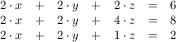If you try to triangulate this SOE—by hand or with your solution from exercise 467you arrive at an intermediate matrix all of whose equations start with 0: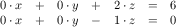Exercise 468. Modify triangulate from exercise 467 so that it signals an error if it encounters an SOE whose leading coefficients are all 0.

After we obtain a triangular system of equations such as (*) in exercise 463, we can solve the equations, one at a time. In our specific example, the last equation says that z is 2. Equipped with this knowledge, we can eliminate z from the second equation through a substitution:Doing so, in turn, determines the value for y: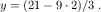Now that we have z = 2 and y = 1, we can plug these values into the first equation: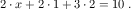This yields another equation in a single variable, which we solve like this: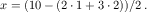This finally yields a value for x and thus the complete solution for the entire SOE.

Exercise 469. Design the solve function. It consumes triangular SOEs and produces a solution.

Hint Use structural recursion for the design. Start with the design of a function that solves a single linear equation in n+1 variables, given a solution for the last n variables. In general, this function plugs in the values for the rest of the left-hand side, subtracts the result from the right-hand side, and divides by the first coefficient. Experiment with this suggestion and the above examples.

Challenge Use an existing abstraction and lambda to design solve.

Exercise 470. Define gauss, which combines the triangulate function from exercise 468 and the solve function from exercise 469.

### 29Algorithms that Backtrack

Problem solving doesn’t always progress along some straight line. Sometimes we may follow one approach and discover that we are stuck because we took a wrong turn. One obvious option is to backtrack to the place where we made the fateful decision and to take a different turn. Some algorithms work just like that. This chapter presents two instances. The first section deals with an algorithm for traversing graphs. The second one is an extended exercise that uses backtracking in the context of a chess puzzle.

#### 29.1Traversing Graphs

Graphs are ubiquitous in our world and the world of computing. Imagine a group of people, say, the students in your school. Write down all the names, and connect the names of those people who know each other. You have just created your first undirected graph.

Now take a look at figure 168, which displays a small directed graph. It consists of seven nodes—the circled letters—and nine edges—the arrows. The graph may represent a small version of an email network. Imagine a company and all the emails that go back and forth. Write down the email addresses of all employees. Then, address by address, draw an arrow from the address to all those addresses to whom the owner sends emails during a week. This is how you would create the directed graph in figure 168, though it might end up looking much more complex, almost impenetrable.Figure 168: A directed graph

In general, a graph consists of a collection of nodes and a collection of edges, which connect nodes. In a directed graph, the edges represent one-way connections between the nodes; in an undirected graph, the edges represent two-way connections between the nodes. In this context, the following is a common type of problem:Social scientists use such algorithms to figure out the power structure in a company. Similarly they use such graphs to predict the probable activities of people, even without knowledge of the content of their emails.

Sample Problem Design an algorithm that proposes a way to introduce one person to another in a directed email graph for a large company. The program consumes a directed graph representing established email connections and two email addresses. It returns a sequence of email addresses that connect the first email with the second.

Mathematical scientists call the desired sequence a path.

Figure 168 makes the sample problem concrete. For example, you may wish to test whether the program can find a path from C to D. This particular path consists of the origination node C and the destination node D. In contrast, if you wish to connect E with D, there are two paths:
• send email from E to F and then to D.

• send it from E to C and then to D.

Sometimes it is impossible to connect two nodes with a path. In the graph of figure 168, you cannot move from C to G by following the arrows.

Looking at figure 168 you can easily figure out how to get from one node to another without thinking much about how you did it. So imagine for a moment that the graph in figure 168 is a large park. Also imagine someone says you are located at E and you need to get to G. You can clearly see two paths, one leading to C and another one leading to F. Follow the first one and make sure to remember that it is also possible to get from E to F. Now you have a new problem, namely, how to get from C to G. The key insight is that this new problem is just like the original problem; it asks you to find a path from one node to another. Furthermore, if you can solve the problem, you know how to get from E to Gjust add the step from E to C. But there is no path from C to G. Fortunately, you remember that it is also possible to go from E to F, meaning you can backtrack to some point where you have a choice to make and restart the search from there.

Now let’s design this algorithm in a systematic manner. Following the general design recipe, we start with a data analysis. Here are two compact list-based representations of the graph in figure 168:
 (define sample-graph '((A (B E)) (B (E F)) (C (D)) (D ()) (E (C F)) (F (D G)) (G ())))

 (define sample-graph '((A B E) (B E F) (C D) (D) (E C F) (F D G) (G)))
Both contain one list per node. Each of these lists starts with the name of a node followed by its (immediate) neighbors, that is, nodes reachable by following a single arrow. The two differ in how they connect the (name of the) node and its neighbors: the left one uses list while the right one uses cons. For example, the second list represents node B with its two outgoing edges to E and F in figure 168. On the left 'B is the first name on a two-element list; on the right it is the first name on a three-element list.

Exercise 471. Translate one of the above definitions into proper list form using list and proper symbols.

The data representation for nodes is straightforward:

; A Node is a Symbol.

Formulate a data definition to describe the class of all Graph representations, allowing an arbitrary number of nodes and edges. Only one of the above representations has to belong to Graph.

Design the function neighbors. It consumes a Node n and a Graph g and produces the list of immediate neighbors of n in g.

Using your data definitions for Node and Graphregardless of which one you chose, as long as you also designed neighborswe can now formulate a signature and a purpose statement for find-path, the function that searches a path in a graph:
 ; Node Node Graph -> [List-of Node] ; finds a path from origination to destination in G (define (find-path origination destination G) '())
What this header leaves open is the exact shape of the result. It implies that the result is a list of nodes, but it does not say which nodes it contains.

To appreciate this ambiguity and why it matters, let’s study the examples from above. In ISL+, we can now formulate them like this:
 (find-path 'C 'D sample-graph) (find-path 'E 'D sample-graph) (find-path 'C 'G sample-graph)
The first call to find-path must return a unique path, the second one must choose one from two, and the third one must signal that there is no path from 'C to 'G in sample-graph. Here are two possibilities, then, on how to construct the return value:
• The result of the function consists of all nodes leading from the origination node to the destination node, including those two.It is easy to imagine others, such as skipping either of the two given nodes. In this case, an empty path could be used to express the lack of a path between two nodes.

• Alternatively, since the call itself already lists two of the nodes, the output could mention only the “interior” nodes of the path. Then the answer for the first call would be '() because 'D is an immediate neighbor of 'C. Of course, '() could then no longer signal failure.

Concerning the lack-of-a-path issue, we must choose a distinct value for signaling this notion. Because #false is distinct, is meaningful, and works in either case, we opt for it. As for the multiple-paths issue, we postpone making a choice for now and list both possibilities in the example section:
 ; A Path is a [List-of Node]. ; interpretation The list of nodes specifies a sequence ; of immediate neighbors that leads from the first ; Node on the list to the last one. ; Node Node Graph -> [Maybe Path] ; finds a path from origination to destination in G ; if there is no path, the function produces #false (check-expect (find-path 'C 'D sample-graph) '(C D)) (check-member-of (find-path 'E 'D sample-graph) '(E F D) '(E C D)) (check-expect (find-path 'C 'G sample-graph) #false) (define (find-path origination destination G) #false)

Our next design step is to understand the four essential pieces of the function: the “trivial problem” condition, a matching solution, the generation of a new problem, and the combination step. The above discussion of the search process and the analysis of the three examples suggest answers:
1. If the two given nodes are directly connected with an arrow in the given graph, the path consists of just these two nodes. But there is an even simpler case, namely, when the origination argument of find-path is equal to its destination.

2. In that second case, the problem is truly trivial and the matching answer is (list destination).

3. If the arguments are different, the algorithm must inspect all immediate neighbors of origination and determine whether there is a path from any one of those to destination. In other words, picking one of those neighbors generates a new instance of the “find a path” problem.

4. Finally, once the algorithm has a path from a neighbor of origination to destination, it is easy to construct a complete path from the former to the latter—just add the origination node to the list.

From a programming perspective, the third point is critical. Since a node can have an arbitrary number of neighbors, the “inspect all neighbors” task is too complex for a single primitive. We need an auxiliary function that consumes a list of nodes and generates a new path problem for each of them. Put differently, the function is a list-oriented version of find-path.

Let’s call this auxiliary function find-path/list and let’s formulate a wish for it:
 ; [List-of Node] Node Graph -> [Maybe Path] ; finds a path from some node on lo-originations to ; destination; otherwise, it produces #false (define (find-path/list lo-originations destination G) #false)
Using this wish, we can fill in the generic template for generative-recursive functions to get a first draft of find-path:
 (define (find-path origination destination G) (cond [(symbol=? origination destination) (list destination)] [else (... origination ... ...(find-path/list (neighbors origination G) destination G) ...)]))
It uses the neighbors from exercise 471 and the wish-list function find-path/list and otherwise uses the answers to the four questions about generative recursive functions.

The rest of the design process is about details of composing these functions properly. Consider the signature of find-path/list. Like find-path, it produces [Maybe Path]. That is, if it finds a path from any of the neighbors, it produces this path; otherwise, if none of the neighbors is connected to destination, the function produces #false. Hence the answer of find-path depends on the kind of result that find-path/list produces, meaning the code must distinguish the two possible answers with a cond expression:
 (define (find-path origination destination G) (cond [(symbol=? origination destination) (list destination)] [else (local ((define next (neighbors origination G)) (define candidate (find-path/list next destination G))) (cond [(boolean? candidate) ...] [(cons? candidate) ...]))]))
The two cases reflect the two kinds of answers we might receive: a Boolean or a list. In the first case, find-path/list cannot find a path from any neighbor to destination, meaning find-path itself cannot construct such a path either. In the second case, the auxiliary function found a path, but find-path must still add origination to the front of this path because candidate starts with one of origination’s neighbors, not origination itself as agreed upon above.

 ; Node Node Graph -> [Maybe Path] ; finds a path from origination to destination in G ; if there is no path, the function produces #false (define (find-path origination destination G) (cond [(symbol=? origination destination) (list destination)] [else (local ((define next (neighbors origination G)) (define candidate (find-path/list next destination G))) (cond [(boolean? candidate) #false] [else (cons origination candidate)]))])) ; [List-of Node] Node Graph -> [Maybe Path] ; finds a path from some node on lo-Os to D ; if there is no path, the function produces #false (define (find-path/list lo-Os D G) (cond [(empty? lo-Os) #false] [else (local ((define candidate (find-path (first lo-Os) D G))) (cond [(boolean? candidate) (find-path/list (rest lo-Os) D G)] [else candidate]))]))

Figure 169: Finding a path in a graph

Figure 169 contains the complete definition of find-path. It also contains a definition of find-path/list, which processes its first argument via structural recursion. For each node in the list, find-path/list uses find-path to check for a path. If find-path indeed produces a path, that path is its answer. Otherwise, find-path/list backtracks.

Note Trees discusses backtracking in the structural world. A particularly good example is the function that searches blue-eyed ancestors in a family tree. When the function encounters a node, it first searches one branch of the family tree, say the father’s, and if this search produces #false, it searches the other half. Since graphs generalize trees, comparing this function with find-path is an instructive exercise. End

Lastly, we need to check whether find-path produces an answer for all possible inputs. It is relatively easy to check that, when given the graph in figure 168 and any two nodes in this graph, find-path always produces some answer. Stop! Solve the next exercise before you read on.

Exercise 472. Test find-path. Use the function to find a path from 'A to 'G in sample-graph. Which one does it find? Why?

Design test-on-all-nodes, a function that consumes a graph g and determines whether there is a path between any pair of nodes.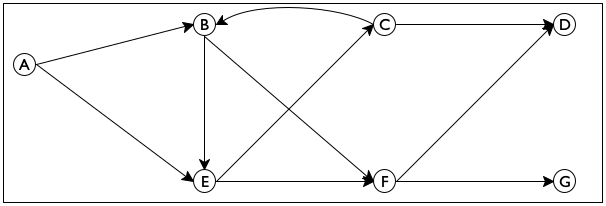Figure 170: A directed graph with cycle

For other graphs, however, find-path may not terminate for certain pairs of nodes. Consider the graph in figure 170.

Stop! Define cyclic-graph to represent the graph in this figure.

Compared to figure 168, this new graph contains only one extra edge, from C to B. This seemingly small addition, though, allows us to start a search in a node and to return to the same node. Specifically, it is possible to move from B to E to C and back to B. Indeed, when find-path is applied to 'B, 'D, and this graph, it fails to stop, as a hand-evaluation confirms:
 (find-path 'B 'D cyclic-graph) == .. (find-path 'B 'D cyclic-graph) .. == .. (find-path/list (list 'E 'F) 'D cyclic-graph) .. == .. (find-path 'E 'D cyclic-graph) .. == .. (find-path/list (list 'C 'F) 'D cyclic-graph) .. == .. (find-path 'C 'D cyclic-graph) .. == .. (find-path/list (list 'B 'D) 'D cyclic-graph) .. == .. (find-path 'B 'D cyclic-graph) ..
The hand-evaluation shows that after seven applications of find-path and find-path/list, ISL+ must evaluate the exact same expression that it started with.You know only one exception to this rule: random. Since the same input triggers the same evaluation for any function, find-path does not terminate for these inputs.

In summary, the termination argument goes like this. If some given graph is free of cycles, find-path produces some output for any given inputs. After all, every path can only contain a finite number of nodes, and the number of paths is finite, too. The function therefore either exhaustively inspects all solutions starting from some given node or finds a path from the origination to the destination node. If, however, a graph contains a cycle, that is, a path from some node back to itself, find-path may not produce a result for some inputs.

The next part presents a program design technique that addresses just this kind of problem. In particular, it presents a variant of find-path that can deal with cycles in a graph.

Exercise 473. Test find-path on 'B, 'C, and the graph in figure 170. Also use test-on-all-nodes from exercise 472 on this graph.

Exercise 474. Redesign the find-path program as a single function.

Exercise 475. Redesign find-path/list so that it uses an existing list abstraction from figures 95 and 96 instead of explicit structural recursion. Hint Read the documentation for Racket’s ormap. How does it differ from ISL+’s ormap function? Would the former be helpful here?

Note on Data Abstraction You may have noticed that the find-path function does not need to know how Graph is defined. As long as you provide a correct neighbors function for Graph, find-path works perfectly fine. In short, the find-path program uses data abstraction.

As Abstraction says, data abstraction works just like function abstraction. Here you could create a function abstract-find-path, which would consume one more parameter than find-path: neighbors. As long as you always handed abstract-find-path a graph G from Graph and the matching neighbors function, it would process the graph properly. While the extra parameter suggests abstraction in the conventional sense, the required relationship between two of the parameters—G and neighborsreally means that abstract-find-path is also abstracted over the definition of Graph. Since the latter is a data definition, the idea is dubbed data abstraction.

When programs grow large, data abstraction becomes a critical tool for the construction of a program’s components. The next volume in the How to Design series addresses this idea in depth; the next section illustrates the idea with another example. End

Exercise 476. Finite State Machines poses a problem concerning finite state machines and strings but immediately defers to this chapter because the solution calls for generative recursion. You have now acquired the design knowledge needed to tackle the problem.

Design the function fsm-match. It consumes the data representation of a finite state machine and a string. It produces #true if the sequence of characters in the string causes the finite state machine to transition from an initial state to a final state.

Since this problem is about the design of generative recursive functions, we provide the essential data definition and a data example:
 (define-struct transition [current key next]) (define-struct fsm [initial transitions final]) ; An FSM is a structure: ;   (make-fsm FSM-State [List-of 1Transition] FSM-State) ; A 1Transition is a structure: ;   (make-transition FSM-State 1String FSM-State) ; An FSM-State is String. ; data example: see exercise 109 (define fsm-a-bc*-d (make-fsm "AA" (list (make-transition "AA" "a" "BC") (make-transition "BC" "b" "BC") (make-transition "BC" "c" "BC") (make-transition "BC" "d" "DD")) "DD"))
The data example corresponds to the regular expression a (b|c)* d. As mentioned in exercise 109, "acbd", "ad", and "abcd" are examples of acceptable strings; "da", "aa", or "d" do not match.

In this context, you are designing the following function:
 ; FSM String -> Boolean ; does an-fsm recognize the given string (define (fsm-match? an-fsm a-string) #false)
Hint Design the necessary auxiliary function locally to the fsm-match? function. In this context, represent the problem as a pair of parameters: the current state of the finite state machine and the remaining list of 1Strings.

 ; [List-of X] -> [List-of [List-of X]] ; creates a list of all rearrangements of the items in w (define (arrangements w) (cond [(empty? w) '(())] [else (foldr (lambda (item others) (local ((define without-item (arrangements (remove item w))) (define add-item-to-front (map (lambda (a) (cons item a)) without-item))) (append add-item-to-front others))) '() w)])) (define (all-words-from-rat? w) (and (member (explode "rat") w) (member (explode "art") w) (member (explode "tar") w))) (check-satisfied (arrangements '("r" "a" "t")) all-words-from-rat?)

Figure 171: A definition of arrangements using generative recursion

Exercise 477. Inspect the function definition of arrangements in figure 171. The figure displays a generative-recursive solution of the extended design problem covered by Word Games, the Heart of the Problem, namelyWe thank Mark Engelberg for suggesting this exercise.

given a word, create all possible rearrangements of the letters.

The extended exercise is a direct guide to the structurally recursive design of the main function and two auxiliaries, where the design of the latter requires the creation of two more helper functions. In contrast, figure 171 uses the power of generative recursion—plus foldr and mapto define the same program as a single function definition.

Explain the design of the generative-recursive version of arrangements. Answer all questions that the design recipe for generative recursion poses, including the question of termination.

Does arrangements in figure 171 create the same lists as the solution of Word Games, the Heart of the Problem?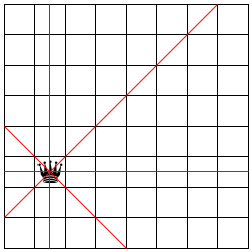Figure 172: A chess board with a single queen and the positions it threatens

#### 29.2Project: Backtracking

The n queens puzzle is a famous problem from the world of chess that also illustrates the applicability of backtracking in a natural way. For our purposes, a chess board is a grid of n by n squares. The queen is a game piece that can move in a horizontal, vertical,We thank Mark Engelberg for his reformulation of this section. or diagonal direction arbitrarily far without “jumping” over another piece. We say that a queen threatens a square if it is on the square or can move to it. Figure 172 illustrates the notion in a graphical manner. The queen is in the second column and sixth row. The solid lines radiating out from the queen go through all those squares that are threatened by the queen.

The classical queens problem is to place 8 queens on an 8 by 8 chess board such that the queens on the board don’t threaten each other. Computer scientists generalize the problem and ask whether it is possible to place n queens on a n by n, chess board such that the queens don’t pose a threat to each other.

For n = 2, the complete puzzle obviously has no solution. A queen placed on any of the four squares threatens all remaining squares.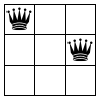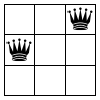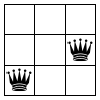Figure 173: Three queen configurations for a 3 by 3 chess board

There is also no solution for n = 3. Figure 173 presents all different placements of two queens, that is, solutions for k = 3 and n = 2. In each case, the left queen occupies a square in the left column while a second queen is placed in one of two squares that the first one does not threaten. The placement of a second queen threatens all remaining, unoccupied squares, meaning it is impossible to place a third queen.

Exercise 478. You can also place the first queen in all squares of the top-most row, the right-most column, and the bottom-most row. Explain why all of these solutions are just like the three scenarios depicted in figure 173.

This leaves the central square. Is it possible to place even a second queen after you place one on the central square of a 3 by 3 board?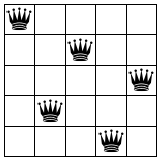Figure 174: Solutions for the n queens puzzle for 4 by 4 and 5 by 5 boards

Figure 174 displays two solutions for the n queens puzzle: the left one is for n = 4, the right one for n = 5. The figure shows how in each case a solution has one queen in each row and column, which makes sense because a queen threatens the entire row and column that radiate out from its square.

Now that we have conducted a sufficiently detailed analysis, we can proceed to the solution phase. The analysis suggests several ideas:

1. The problem is about placing one queen at a time. When we place a queen on a board, we can mark the corresponding rows, columns, and diagonals as unusable for other queens.

2. For another queen, we consider only nonthreatened spots.

3. Just in case this first choice of a spot leads to problems later, we remember what other squares are feasible for placing this queen.

4. If we are supposed to place a queen on a board but no safe squares are left, we backtrack to a previous point in the process where we chose one square over another and try one of the remaining squares.

In short, this solution process is like the “find a path” algorithm.

Moving from the process description to a designed algorithm clearly calls for two data representations: one for the chess boards and one for positions on the board. Let’s start with the latter:
 (define QUEENS 8) ; A QP is a structure: ;   (make-posn CI CI) ; A CI is an N in [0,QUEENS). ; interpretation (make-posn r c) denotes the square at ; the r-th row and c-th column
After all, the chess board basically dictates the choice.

The definition for CI could use [1,QUEENS] instead of [0, QUEENS), but the two definitions are basically equivalent and counting up from 0 is what programmers do. Similarly, the so-called algebraic notation for chess positions uses the letters 'a through 'h for one of the board’s dimensions, meaning QP could have used CIs and such letters. Again, the two are roughly equivalent and with natural numbers it is easier in ISL+ to create many positions than with letters.

Exercise 479. Design the threatening? function. It consumes two QPs and determines whether queens placed on the two respective squares would threaten each other.

Domain Knowledge (1) Study figure 172. The queen in this figure threatens all squares on the horizontal, the vertical, and the diagonal lines. Conversely, a queen on any square on these lines threatens the queen.

(2) Translate your insights into mathematical conditions that relate the squares’ coordinates to each other. For example, all squares on a horizontal have the same y-coordinate. Similarly, all squares on one diagonal have coordinates whose sums are the same. Which diagonal is that? For the other diagonal, the differences between the two coordinates remain the same. Which diagonal does this idea describe?

Hint Once you have figured out the domain knowledge, formulate a test suite that covers horizontals, verticals, and diagonals. Don’t forget to include arguments for which threatening? must produce #false.

Exercise 480. Design render-queens. The function consumes a natural number n, a list of QPs, and an Image. It produces an image of an n by n chess board with the given image placed according to the given QPs.

You may wish to look for an image for a chess queen on-line or create a simplistic one with the available image functions.

As for a data representation for Boards, we postpone this step until we know how the algorithm implements the process. Doing so is another exercise in data abstraction. Indeed, a data definition for Board isn’t even necessary to state the signature for the algorithm proper:
 ; N -> [Maybe [List-of QP]] ; finds a solution to the n queens problem ; data example: [List-of QP] (define 4QUEEN-SOLUTION-2 (list  (make-posn 0 2) (make-posn 1 0) (make-posn 2 3) (make-posn 3 1))) (define (n-queens n) #false)
The complete puzzle is about finding a placement for n queens on an n by n chess board. So clearly, the algorithm consumes nothing else but a natural number, and it produces a representation for the n queen placements—if a solution exists. The latter can be represented with a list of QPs, which is why we choose

; [List-of QP] or #false

as the result. Naturally, #false represents the failure to find a solution.

The next step is to develop examples and to formulate them as tests. We know that n-queens must fail when given 2 or 3. For 4, there are two solutions with real boards and four identical queens. Figure 174 shows one of them, on the left, and the other one is this: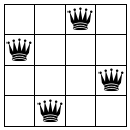In terms of data representations, however, there are many different ways to represent these two images. Figure 175 sketches some. Fill in the rest.

 ; N -> [Maybe [List-of QP]] ; finds a solution to the n queens problem (define 0-1 (make-posn 0 1)) (define 1-3 (make-posn 1 3)) (define 2-0 (make-posn 2 0)) (define 3-2 (make-posn 3 2)) (check-member-of (n-queens 4) (list 0-1 1-3 2-0 3-2) (list 0-1 1-3 3-2 2-0) (list 0-1 2-0 1-3 3-2) (list 0-1 2-0 3-2 1-3) (list 0-1 3-2 1-3 2-0) (list 0-1 3-2 2-0 1-3) ... (list 3-2 2-0 1-3 0-1)) (define (n-queens n) (place-queens (board0 n) n))

Figure 175: Solutions for the 4 queens puzzle

Exercise 481. The tests in figure 175 are awful. No real-world programmer ever spells out all these possible outcomes.

One solution is to use property testing again. Design the n-queens-solution? function, which consumes a natural number n and produces a predicate on queen placements that determines whether a given placement is a solution to an n queens puzzle:
• A solution for an n queens puzzle must have length n.

• A QP on such a list may not threaten any other, distinct QP.

Once you have tested this predicate, use it and check-satisfied to formulate the tests for n-queens.

An alternative solution is to understand the lists of QPs as sets. If two lists contain the same QPs in different order, they are equivalent as the figure suggests. Hence you could formulate the test for n-queens as
 ; [List-of QP] -> Boolean ; is the result equal [as a set] to one of two lists (define (is-queens-result? x) (or (set=? 4QUEEN-SOLUTION-1 x) (set=? 4QUEEN-SOLUTION-2 x)))
Design the function set=?. It consumes two lists and determines whether they contain the same items—regardless of order.

Exercise 482. The key idea to is to design a function that places n queens on a chess board that may already contain some queens:
 ; Board N -> [Maybe [List-of QP]] ; places n queens on board; otherwise, returns #false (define (place-queens a-board n) #false)
Figure 175 already refers to this function in the definition of n-queens.

Design the place-queens algorithm. Assume you have the following functions to deal with Boards:
 ; N -> Board ; creates the initial n by n board (define (board0 n) ...) ; Board QP -> Board ; places a queen at qp on a-board (define (add-queen a-board qp) a-board) ; Board -> [List-of QP] ; finds spots where it is still safe to place a queen (define (find-open-spots a-board) '())
The first function is used in figure 175 to create the initial board representation for place-queens. You will need the other two to describe the generative steps for the algorithm.

You cannot confirm yet that your solution to the preceding exercise works because it relies on an extensive wish list. It calls for a data representation of Boards that supports the three functions on the wish list. This, then, is your remaining problem.

Exercise 483. Develop a data definition for Board and design the three functions specified in exercise 482. Consider the following ideas:
• a Board collects those positions where a queen can still be placed;

• a Board contains the list of positions where a queen has been placed;

• a Board is a grid of n by n squares, each possibly occupied by a queen. Use a structure with three fields to represent a square: one for x, one for y, and a third one saying whether the square is threatened.

Use one of the above ideas to solve this exercise.

Challenge Use all three ideas to come up with three different data representations of Board. Abstract your solution to exercise 482 and confirm that it works with any of your data representations of Board.

### 30Summary

This fifth part of the book introduces the idea of eureka! into program design. Unlike the structural design of the first four parts, eureka! design starts from an idea of how the program should solve a problem or process data that represents a problem. Designing here means coming up with a clever way to call a recursive function on a new kind of problem that is like the given one but simpler.

Keep in mind that while we have dubbed it generative recursion, most computer scientists refer to these functions as algorithms.

Once you have completed this part of the book, you will understand the following about the design of generative recursion:
1. The standard outline of the design recipe remains valid.

2. The major change concerns the coding step. It introduces four new questions on going from the completely generic template for generative recursion to a complete function. With two of these questions, you work out the “trivial” parts of the solution process; and with the other two you work out the generative solution step.

3. The minor change is about the termination behavior of generative recursive functions. Unlike structurally designed functions, algorithms may not terminate for some inputs. This problem might be due to inherent limitations in the idea or the translation of the idea into code. Regardless, the future reader of your program deserves a warning about potentially “bad” inputs.

You will encounter some simple or well-known algorithms in your real-world programming tasks, and you will be expected to cope. For truly clever algorithms, software companies employ highly paid specialists, domain experts, and mathematicians to work out the conceptual details before they ask programmers to turn the concepts into programs. You must also be prepared for this kind of task, and the best preparation is practice.